# Fixed point vs. Floating Point

When an analogue audio signal is converted to a digital representation, the value of the level for each sample is rounded to the nearest quantisation step (because a digital audio system does not have an infinite resolution). I’ve talked about this in detail in a past posting.

When a sample value in a digital audio stream is stored or transmitted inside a piece of audio equipment or software, one of the choices the engineer can make is whether the value should be represented using a fixed point or a floating point system. These are related, but fundamentally different, and they have some effects on the audio signal that may be audible if you’re not careful…

Let’s lay down some basic points to start. We’ll say the following:

• Audio is a kind of AC signal that has a level that can vary between two values.
• For now, we’ll say that the limits on the range of values is -1 and +1, and it can be anything in between.
• We’re going to divide up that range into some finite number of steps and round the actual signal value to the closest usable value (we’re not talking about dither today)
• The value will be stored as a binary number somehow

The question that we’ll look at here is exactly how that binary value represents the number, and a little of what that means to the audio signal.

## Fixed Point Representation

The simplest way to represent the value is to divide the total range from the minimum to the maximum number into an equal number of steps, and round the signal’s value to the closest step. This is a really generalised description of a “fixed point” system.

For example, if we have a 3-bit number to play with, we’ll take the first bit and use that one to represent the + or – portion of the value (where 0 means “+” and 1 means “-“). For values from 0 up to (just under) the positive maximum, the other 2 bits are used to just count the steps, from 000 up to 011. The negative values start at the bottom and work their way up to 1 step below 0, from 100 to 111. This can be seen in Figure 1.Figure 1: A simplified representation of the use of quantisation steps in a 3-bit fixed point system.

If you look carefully at Figure 1, you’ll see that there is one extra negative step, since one of the positive steps is used to represent the value 0 in the middle. This means that, if the signal is symmetrical, then we will wind up using all of the possible quantisation values except for the bottom one (just like I’ve shown in the plot), however, for the rest of this discussion, we’ll be working with numbers that are so big that this one step doesn’t really matter, so I won’t mention it again.

If we are using a 3-bit number to represent the value, then we have a total number of 23 quantisation steps: 8 of them. Each time we add one more bit, we double the number of steps. So, for a 16-bit sample, we have 216, or 65,536 possible quantisation values. For a 24-bit sample, we have 224, or 16,777,216 steps.

By increasing the number of bits in the number, we don’t change the level (it still has a range of -1 to +1), we’re just increasing the resolution that we have to make the measurement. The higher the resolution, the lower the error, and so the lower the level of distortion (if we don’t dither) or noise (if we do) relative to the signal.

If you have a fixed-point system, and you want to calculate the difference in level between the maximum signal level and the noise floor, then you can use a somewhat simplified equation, shown below:

Dynamic Range In dB ≈ 6 * nBits – 3

As I said, this is simplified due to some rounding to keep the numbers nice, but the general idea is that you have a doubling of dynamic range for every extra bit (therefore 6 dB per bit) and you lose 3 dB for the (TPDF) dither (but that’s better than not having the dither and having distortion instead). If you wanted to do it properly, then you can use this math instead:

Dynamic Range In dB ≈ 20*log10(2nBits) – 20*log10(sqrt(2))

So, if you have a 16-bit fixed point system, you have about 93 dB of range from the loudest signal to the noise floor. If you have a 24-bit system, it’s about 141 dB.

Remember that the noise floor is constant (I’m assuming it’s dithered), so as the signal level drops below maximum the current signal to noise ratio will drop by the same amount. Therefore, if your signal is 12 dB below maximum (or -12 dB FS, which means “12 decibels below Full Scale”), then the SNR in a 16-bit system is 93 – 12 = 81 dB.

If that last paragraph didn’t make complete sense, go back and read it again, because it’ll come back later…

Fixed point is a good system for conversion of an audio signal from and to analogue, but if you’re doing some really serious processing, it might not work out so well. This is due to two primary reasons:

• If your signal is going to outside the range, it will clip at the maximum positive or the minimum negative value because fixed point is not designed to exceed its range.
• If the signal is going to be reduced to a very low level somewhere in your proceeding (say, inside a biquad, for example) then you might need a LOT of bits to keep the noise floor low enough when the signal level is brought back up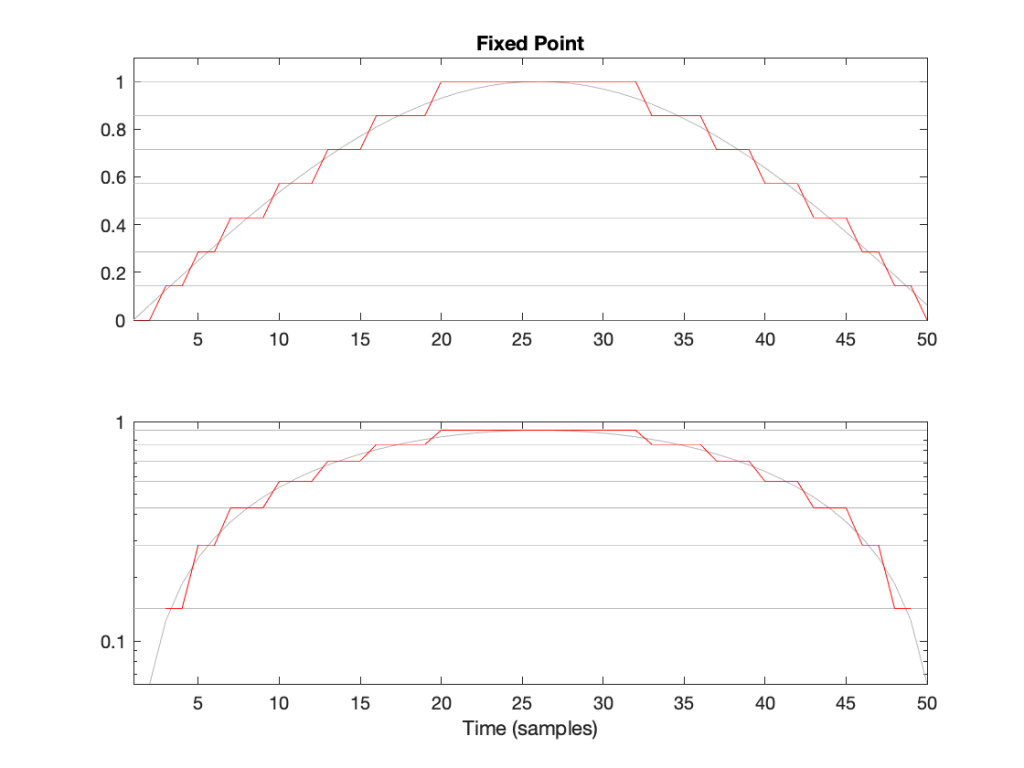Figure 2: The first half of a sine wave (in grey) quantised (without dither) in a simplified 4-bit fixed point system. (I’ve actually cheated a bit and just made 8 equally-spaced steps from 0 to 1 unlike the version shown in Figure 1.) The two plots show identical data, but the bottom plot has a logarithmically-scaled Y-axis.

As can be seen in Figure 2, the equally-spaced steps in a fixed point world mean that the quantisation error is always between -0.5 and 0.5 of a step (a “Least Significant Bit” or LSB), regardless of the level of the signal.

## Floating Point Representation

There is another way to use the bits to represent the signal value. This is to divide the binary “word” into two parts and to do a little math involving some subtraction, multiplication, and an exponent to arrive at the value. Just like in the Fixed Point case, we’ll reserve one bit for the +/- indicator.

Let’s say that we have a 32-bit value to work with. We’ll divide this up into the following:

• 23 bits for the fraction or mantissa, which we’ll abbreviate f
• 8 bits for the exponent, abbreviated e
• 1 bit for the +/- sign (just like in Fixed Point)

We’ll then do the following math:

Sample Value = ± (1 – f) * 2e

We need to know a little extra information:

• because we’re using 23 bits for f, then it can range from 0 to 223-1. In other words, stated mathematically:
0 ≤ 223*f < 223
• because we’re using 8 bits for e, then it has a total range of 28 possible values. In other words it has a range from just over -27 to just under 27. In other words, stated mathematically:
-126 ≤ e ≤ 127
(Note that a couple of possible values are reserved for special purposes, but we won’t talk about those)

This is all a little complicated, but there is a “punch line” to which I’m headed:

Unlike Fixed Point representation, the divisions of the values – the number of steps, and therefore the step sizes – are not the same across the entire scale of possible values. It’s divided into sections, where each section has quantisation steps of equal size, but that step size is dependent on what the value is. In other words the step size changes with the value, but on a coarser scale.

That step size can be calculated as follows:

From 2e to 2e+1, the steps all have an equal size of 2e-fBits where fBits is the number of bits used to express f (in our case, fBits = 23 bits). In other words, we have 2fBits equally-spaced steps in that range.

Therefore, each time the signal value moves (say from just below 0.5 to just above) then the resolution changes, and the higher the value, the smaller the resolution. This is is how Floating Point representation behaves.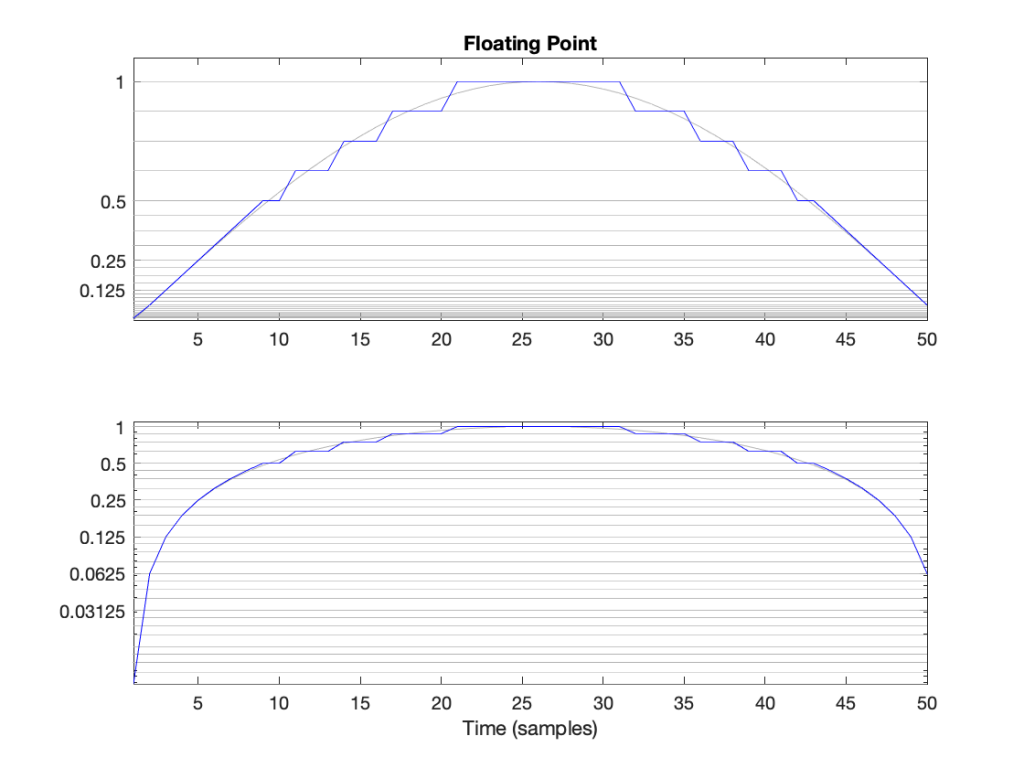Figure 3: The first half of a sine wave (in grey) quantised (without dither) in a simplified floating point system with 2 bits for the fraction. This means that there are 4 equally-spaced steps from (for example) 0.25 to 0.5 or 0.5 to 1. The two plots show identical data, but the bottom plot has a logarithmically-scaled Y-axis.

## Do I care?

Let’s find out.

If I have a signal that has level that has just come up to 1 (or 20), then the resolution of the value (which defines the error, which defines the “distance” in dB to the noise floor) is 2-25 (or 1/33,554,432).* This means that the noise floor is about 150 dB below the signal (20 * log10(1 / 2-25). As the signal level drops to 0.5, the noise floor remains the same, so the signal drops by 6 dB, and the SNR reduces to 150 – 6 = 144 dB.

Then, when we drop just below 0.5, the resolution of the value suddenly changes to 2-26 (or 1/67,108,864) , which means that the noise floor is about 150 dB below the signal (20 * log10(0.5 / 2-26). As the signal drops to 0.25 (-6 dB relative to 0.5), the noise floor remains the same, so the signal drops by 6 dB, and the SNR reduces to 150 – 6 = 144 dB.

Then, when we drop just below 0.25, the resolution of the value suddenly changes to 2-27 (or 1/134,217,728), which means that the noise floor is about 150 dB below the signal (20 * log10(0.25 / 2-27). As the signal drops to 0.25 (-6 dB relative to 0.5), the noise floor remains the same, so the signal drops by 6 dB, and the SNR reduces to 150 – 6 = 144 dB.

Hopefully, by now, you’re seeing a pattern here.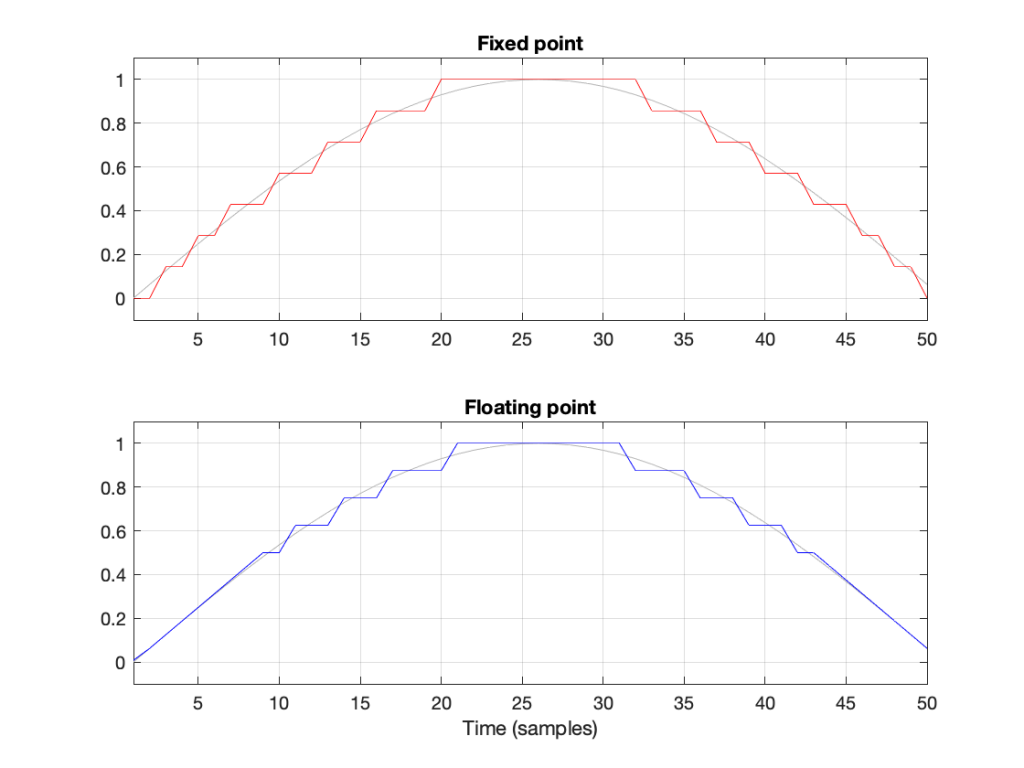Figure 4: Notice that the error of the floating point version is reduced when the signal level (in grey) approaches 0.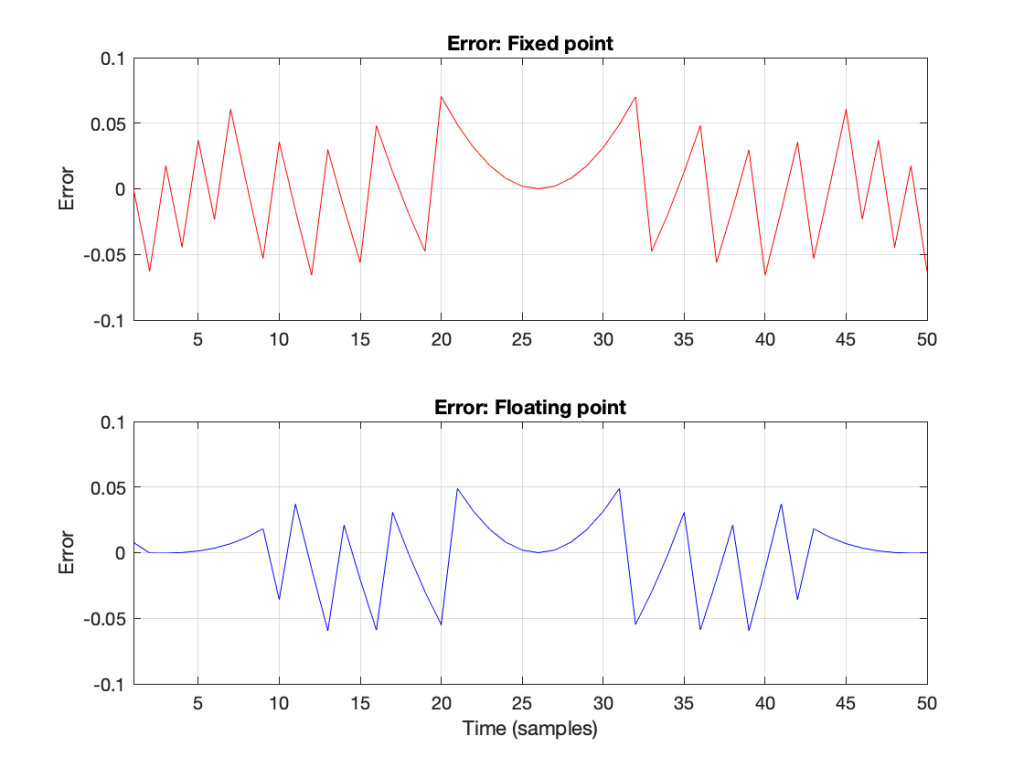Figure 6: The errors from the quantisation shown Figure 5. These are just the original signal subtracted from the quantised signals. Notice that, in Floating Point, the general level of the error is dependent on the level of the signal (it’s smaller on the left and right of the plot) whereas in Fixed Point, the overall level of the error is more constant.

The cool thing is that the pattern would have been the same if I had gone above 1 instead of below it. So, the two things to worry about in Fixed Point (inadequate resolution with (temporarily) low-level signals and clipping when the signal goes outside the range) are not problems in floating point. And, if you have enough bits (32-bit floating point is the standard “single precision” resolution, but 64-bit “double precision” resolution is not uncommon).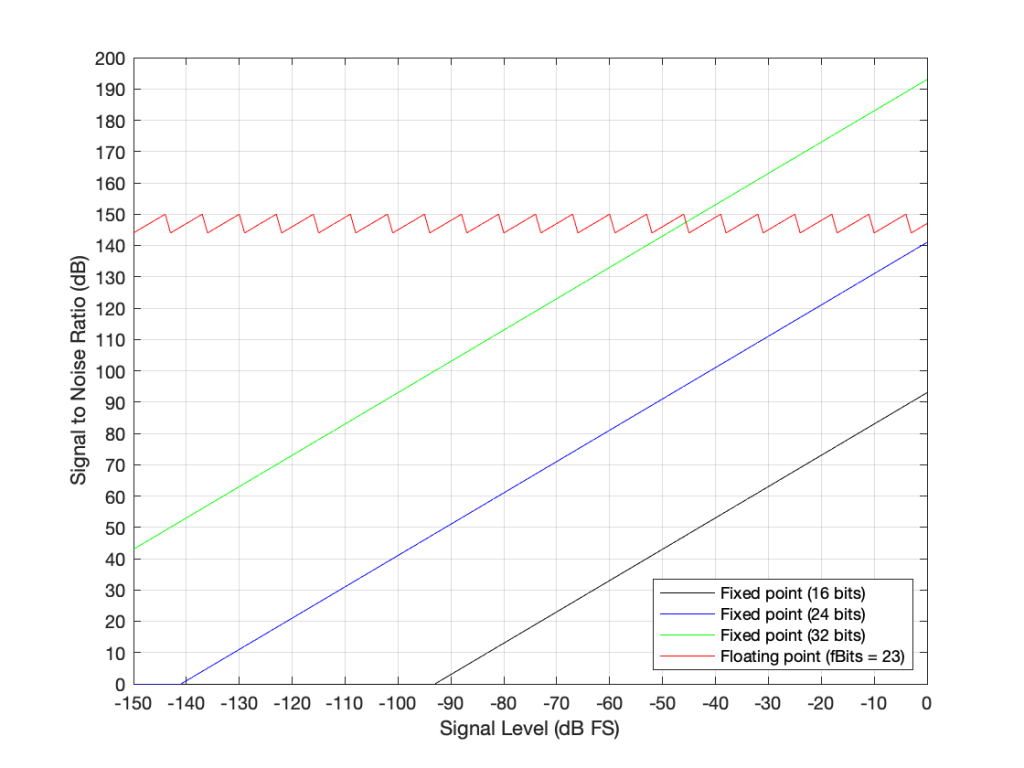Figure 7: The Signal to Noise ratio of four different systems, as a function of the signal level in dB FS.

This is why, in most modern audio systems, you have a fixed-point ADC and a DAC (an Analogue to Digital Converter and a Digital to Analogue converter) at the input and output of your system (because the signal range is pretty well defined, and the dynamic range is more than adequate if you do it right) but the processing on the inside is done in 32-bit or 64-bit floating point (or both, in some devices) so that the engineers have the resolution and the range to play with the signals before getting them ready for the output.

There may be some argument made for a constant noise floor level in a fixed-point system (assuming it’s dithered) over a signal-modulated noise level in a floating-point world (assuming it’s not), however, there are two reasons why this is likely not a real-world issue. The first is that, even in a single-precision floating point system, the worst-case signal to noise ratio is about 144 dB, which is very good. The second is that smart people have already been thinking about dither for floating point systems. If this sounds interesting, you can start reading here

## * Small note for the attentive

You may have noticed what appears to be a mistake in my math in there. First I said:

From 2e to 2e+1, the steps all have an equal size of 2e-fBits where fBits is the number of bits used to express f (in our case, fBits = 23 bits). In other words, we have 2fBits equally-spaced steps in that range.

Then I did the math and said

If I have a signal that has level that has just come up to 1 (or 20), then the resolution of the value (which defines the error, which defines the “distance” in dB to the noise floor) is 2-25 (or 1/133,554,432).

Why did I say 2-25 when maybe I should have said 2-23 ? The reason is that the 223 quantisation levels are located between 1 down to 0.5. If I were to continue the same spacing down to 0, then I would have twice as many quantisation levels, so there would be 224 instead. If I were to continue the spacing all the way down to -1, then there would be twice as many again, or 225.

In other words, a floating point signal ranging from a value of 2-1 to 20 (0.5 to 1) with some number of bits in the fraction that we’re calling fBits will have almost exactly the same signal to noise ratio as a fixed point system that is scaled to range from -1 to 1 with fBits+2.

This would be the same from -20 to -2-1 (-1 to -0.5).

At any other signal value, the quantisation behaviours (and therefore the signal-to-noise ratios) of the two systems will be significantly different.

This is visible in Figure 6 where, when the signal is high (in the middle of the plots), the error level is approximately the same in the 4-bit fixed-point system and the floating point system with 2 bits for the fraction.

Floating Point Numbers posted by Cleve Moler at Mathworks

Floating Point Denormals, Insignificant But Controversial posted by Cleve Moler at Mathworks

# “High-Res” Audio: Part 12: Outputs

This series has flipped back and forth between talking about high resolution audio files & sources and the processing that happens in the equipment when you play it. For this posting, we’re going to deal exclusively with the playback side – regardless of the source content.

I work for a company that makes loudspeakers (among other things). All of the loudspeakers we make use digital signal processing instead of resistors, capacitors, and inductors because that’s the best way to do things these days…

Point 1: This means that our volume control is a gain (a multiplier) that’s applied to the digital signal.

We also make surround processors (most of our customers call them “televisions”) that take a multichannel audio input (these days, this is under the flag of “spatial audio”, but that’s just a new name on an old idea) and distribute the signals to multiple loudspeakers. Consequently, all of our loudspeakers have the same “sensitivity”. This is a measurement of how loud the output is for a given input.

Let’s take one loudspeaker model, Beolab 90, as an example. The sensitivity of this loudspeaker is set to be the same as all other Bang & Olufsen loudspeakers. Originally, this was based on an analogue signal, but has since been converted to digital.

Point 2: Specifically, if you send a 0 dB FS signal into a Beolab 90 set to maximum volume, then it will produce a little over 122 dB SPL at 1 m in a free field (theoretically).

Let’s combine points 1 and 2, with a consideration of bit depth on the audio signal.

If you have a DSP-based loudspeaker with a maximum output of 122 dB SPL, and you play a 16-bit audio signal with nothing but TPDF dither, then the noise floor caused by that dither will be 122 – 93 = 29 dB SPL which is pretty loud. Certainly loud enough for a customer to complain about the noise coming from their loudspeaker.

Now, you might say “but no one would play a CD at maximum volume on that loudspeaker” to which I say two things:

1. I do.
The “Banditen Galop” track from Telarc’s disc called “Ein Straussfest” has enough dynamic range that this is not dangerous. You just get very loud, but very short spikes when the gunshots happen.
2. That’s not the point I’m trying to make anyway…

The point I’m trying to make is that, if Beolab 90 (or any other Bang & Olufsen loudspeaker) used 16-bit DACs, then the noise floor would be 29 dB SPL, regardless of the input signal’s bit depth or dynamic range.

So, the only way to ensure that the DAC (or the bit depth of the signal feeding the DAC) isn’t the source of the noise floor from the loudspeaker is to use more than 16 bits at that point in the signal flow. So, we use a 24-bit DAC, which gives us a (theoretical) noise floor of 122 – 141 = -19 dB SPL. Of course, this is just a theoretical number, since there are no DACs with a 141 dB dynamic range (not without doing some very creative cheating, but this wouldn’t be worth it, since we don’t really need 141 dB of dynamic range anyway).

So, there are many cases where a 24-bit DAC is a REALLY good idea, even though you’re only playing 16-bit recordings.

Similarly, you want the processing itself to be running at a higher resolution than your DAC, so that you can control its (the DAC’s) signal (for example, you want to create the dither in the DSP – not hope that the DAC does it for you. This is why you’ll often see digital signal processing running at floating point (typically 32-bit floating point) or fixed point with a wider bit depth than the DAC.

# “High-Res” Audio: Part 10: The myth of temporal resolution

If you read about high resolution audio – or you talk to some proponents of it, occasionally you’ll hear someone talk about “temporal resolution” or “micro details” or some such nonsense… This posting is just my attempt to convince the world that this belief is a load of horse manure – and that anyone using timing resolution as a reason to use higher sampling rates has no idea what they’re talking about.

Now that I’ve gotten that off my chest, let’s look at why these people could be so misguided in their belief systems…

Many people use the analogy of film to explain sampling. Even I do this – it’s how I introduced aliasing in Part 3 of this series. This is a nice analogy because it uses a known concept (converting movement into a series of still “samples”, frame by frame) to explain a new one. It also has some of the same artefacts, like aliasing, so it’s good for this as well.

The problem is that this is just an analogy – digital audio conversion is NOT the same as film. This is because of the details when you zoom in on a time scale.

Film runs at 24 frames per second (let’s say that’s true, because it’s true enough). This means that the time between on frame of film being shot and the next frame being shot is 1/24th of a second. However, the shutter speed – the time the shutter is open to make each individual photograph is less than 1/24th of a second – possibly much less. Let’s say, for the purposes of this discussion, that it’s 1/100th of a second. This means that, at the start of the frame, the shutter opens, then closes 1/100th of a second later. Then, for about 317/10,000ths of a second, the shutter is closed (1/24 – 1/100 ≈ 317/10,000). Then the process starts again.

In film, if something happened while that shutter was closed for those 317 ten-thousandths of a second, whatever it was that happened will never be recorded. As far as the film is concerned, it never happened.

This is not the way that digital audio works. Remember that, in order to convert an analogue signal into a digital representation, you have to band-limit it first. This ensures (at least in theory…) that there is no signal above the Nyquist frequency that will be encoded as an alias (a different frequency) in the digital domain.

When that low-pass filtering happens, it has an effect in the time domain (it must – otherwise it wouldn’t have an effect in the frequency domain). Let’s look at an example of this…

Let’s say that you have an analogue signal that consists of silence and one almost-infinitely short click that is converted to LPCM digital audio. Remember that this click goes through the anti-aliasing low-pass filter, and then gets sampled at some time. Let’s also say that, by some miracle of universal alignment of planets and stars, that click happened at exactly the same time as the sample was measured (we’ll pretend that this is a big deal and I won’t suggest otherwise for the rest of this posting). The result could look like Figure 1.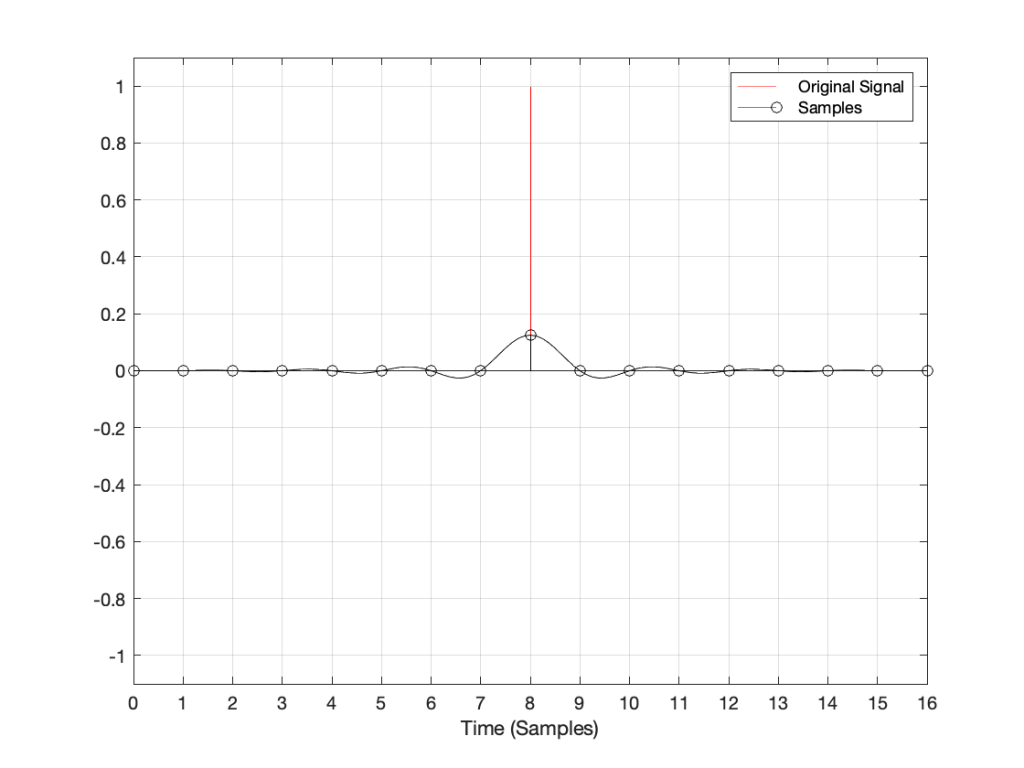Figure 1: A click in the analogue domain (red) sampled and encoded as a digital signal (black circles). The analogue output of the digital system will be the black line

If I zoom in on Figure 1 vertically, it looks like the plot in Figure 2.

There are at least three things to notice in these plots.

• Since the click happened at the same time as a sample, that sample value is high.
• Since the click happened at the same time as a sample, all other sample values are 0.
• Once the digital signal is converted back to analogue later (shown as the black line) the maximum point in the signal will happen at exactly the same time as the click

I won’t talk about the fact that the maximum sample value is lower than the original click yet… we’ll deal with that later.

Now, what would happen if the click did not occur at the same time as the sample time? For example, what if the click happened at exactly the half-way point between two samples? This result is shown in Figure 3.

Notice now that almost all samples have some non-zero value, and notice that the two middle samples (8 and 9) are equal. This means that when the signal is converted to analogue (as is shown with the black line) the time of maximum output is half-way between those two samples – at exactly the same time that the click happened.

Let’s try some more:

I could keep doing this all night, but there’s no point. The message here is, no matter when in time the click happened, the maximum output of the digital signal, after it’s been converted back to analogue, happens at exactly the same time.

But, you ask, what about all that “temporal smearing” – the once-pristine click has been reduced to a long wave that extends in time – both forwards and backwards? Waitaminute… how can the output of the system start a wave before something happened?

## Okay, okay…. calm down.

Firstly, I’ve made this example using only one type of anti-aliasing filter, and only one type of reconstruction filter. The waveforms I’ve shown here are valid examples – but so are other examples… This depends on the details of the filters you use. In this case, I’m using “linear phase” filters which are symmetrical in time. I could have used a different kind of filter that would have looked different – but the maximum point of energy would have occurred at the same time as the click. Because of this temporal symmetry, the output appears to be starting to ring before the input – but that’s only because of the way I plotted it. In reality, there is a constant delay that I have removed before doing the plotting. It’s just a filter, not a time machine.

Secondly, the black line is exactly the same signal you would get if you stayed in the analogue domain and just filtered the click using the two filters I just mentioned (because, in this discussion, I’m not including quantisation error or dither – they have already been discussed as a separate topic…) so the fact that the signal was turned into “digital” in between was irrelevant.

Thirdly, you may still be wondering why the level of the black line is so low compared to the red line. This is because the energy is distributed in time – so, in fact, if you were to listen to these two clicks, they’d sound like they’re the same level. Another way to say it is that the black line shows exactly the same as if the red curve was band-limited. The only thing missing is the upper part of the frequency band. (You may notice that I have not said anything about the actual sampling rate in any of this posting, because it doesn’t matter – the overall effect in the time domain is the same.)

Fourthly, hopefully you are able to see now that an auditory event that happens between two samples is not thrown away in the conversion to digital. Its timing information is preserved – only its frequency is band-limited. If you still don’t believe me, go listen to a digital recording (which is almost all recordings today) of a moving source – anything moving more than 7 mm will do*. If you can hear clicks in the sound as the source moves, then I’m wrong, and the arrival time of the sound is quantising to the closest sample. However, you won’t hear clicks (at least not because the source is moving), so I’m not wrong. Similarly, if digital audio quantised audio events to the nearest sample, an interpolated delay wouldn’t work – and since lots of people use “flanger” and “phaser” effects on their guitar solos with their weekend garage band, then I’m still right…

The conclusion

Hopefully, from now on, if you are having an argument about high resolution audio, and the person you’re arguing with says “but what about the timing information!? It’s lost at 44.1 kHz!” The correct response is to state (as calmly as possible) “BullS#!T!!”

Rant over.

* I said “7 mm” because I’m assuming a sampling rate of 48 kHz, and a speed of sound of 344 m/s. This means that the propagation distance in air is 344/48000 = 0.0071666 m per sample. In other words, if you’re running a 48 kHz signal out of a loudspeaker, the amplitude caused by a sample is 7 mm away when the next sample comes out.

Thought another way, if you have a stereo system, and your left loudspeaker is 7 mm further away from you than your right loudspeaker, at 48 kHz, you can delay the right loudspeaker by 1 sample to re-align the times of arrival of the two signals at the listening position.

On to Part 11

# “High-Res” Audio: Part 8a: Filter resolution (The Setup…)

Almost every audio system has filters or equalisers in it for some reason or another. Originally, equalisers were named that because they were put in on long-distance telephone lines to make the balance of the frequency content more equal. Nowadays, we use equalisers to do things like add bass, or to add more bass.

In the “old days” audio filters were made by building circuits with resistors, capacitors, and inductors: if you choose the relationships between the values of these devices correctly, you can affect the magnitude response as you choose. The problem was production tolerances: if you take two resistors out of the package, and both are supposed to have the same resistance – they’ll be close, but they won’t be identical.

One of the great things about audio filters implemented in a digital system is that you don’t need to worry about variations as a result of production differences. Since digital filters are “just math”, you put the same equation in every device, and you get the same answer for the same input every time. (In the same way that, if I have two calculators on my desk, and I put “2 x 3″ into both of them, and press”=”, I’ll get the same answer on both devices.)

So, to start, let’s talk a little about how a digital filter works. Generally speaking, digital filters work by taking an audio signal, delaying it, changing the level, and adding the result back to the signal itself. Let’s take a simple example, shown in Figure 1.

Let’s say that, to start, we make the gain in that signal flow = 1, and set the delay to equal 1 sample. In this case:

• At a very low frequency, the output of the delay has almost exactly the same value as its input (because 1 sample is a phase difference of almost 0º at a low frequency). When you add a signal to (nearly) itself, you get twice the output – a gain of 6 dB.
• As the frequency of the input goes higher and higher, the delay (of 1 sample) is more and more significant, and therefore its output value gets more and more different from its input value.
• When the input signal’s frequency is 1/2 of the sampling rate, then a delay of 1 sample is equal to a 90º phase shift. When you add a sine wave to itself with a “delay” (actually a “phase shift”) of 90º, the result is a magnitude that is 3 dB higher than the original.
• When the input signal’s frequency is 1/3 of the sampling rate, then a delay of 1 sample is equal to a 120º phase shift. When you add a sine wave to itself with a “delay” (actually a “phase shift”) of 120º, there’s no change in the magnitude (the level).
• When the frequency is 1/2 the sampling rate, then each consecutive sample is 180º out of phase with the previous one, so the sum of the delay and the signal results in complete cancellation, and you get no output at all.

An example of the magnitude response plot of this is shown below in Figure 2.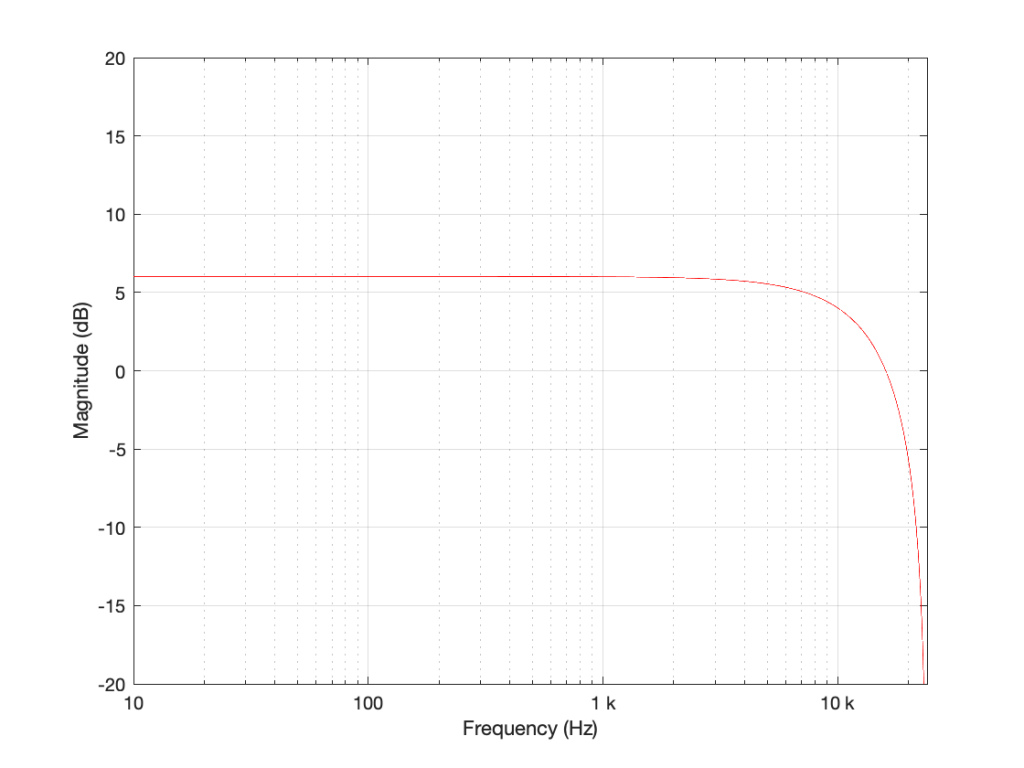Figure 2: The magnitude response of the filter shown in Figure 1 when the delay is set to 1 sample, the gain is set to 1 and Fs=48 kHz.

If we reduce the gain to, say 0.5, then the effect of adding the delayed signal is reduced. The overall shape of the magnitude response is the same, it’s just less, as shown in Figure 3.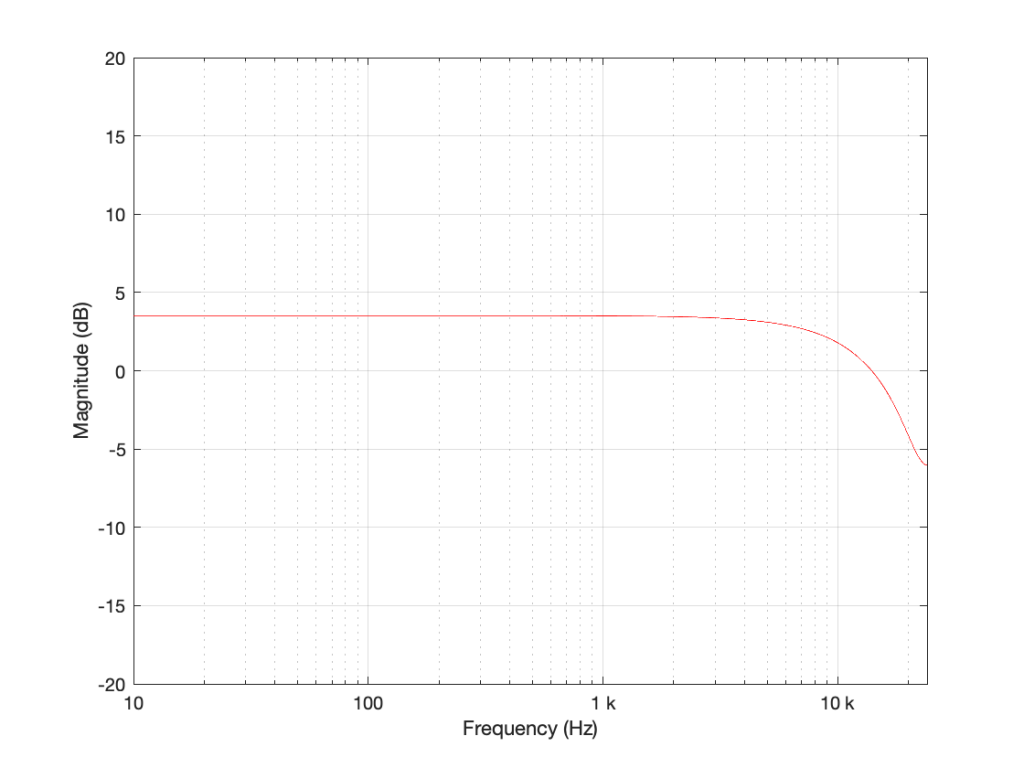Figure 3: The magnitude response of the filter shown in Figure 1 when the delay is set to 1 sample, the gain is set to 0.5 and Fs=48 kHz.

Notice in Figure 3 that the boost in the low end is less, and the dip in the high end is also less than in Figure 2. So, by adjusting the gain on the delayed signal that’s added to the original signal, we can adjust how much this filter is affecting the signal.

What happens when we change the delay? If we make it 2 samples instead of 1, then the phase difference between the output and the input of the delay will be bigger for a given frequency. This also means that the delay will be equivalent to a 180º phase shift at 1/4 Fs (instead of 1/2). Also, at 1/2 Fs, the delay will be equivalent to a 360º phase shift, so the signal adds constructively, just like it does in the low frequencies. So, the resulting magnitude response will look like Figure 4.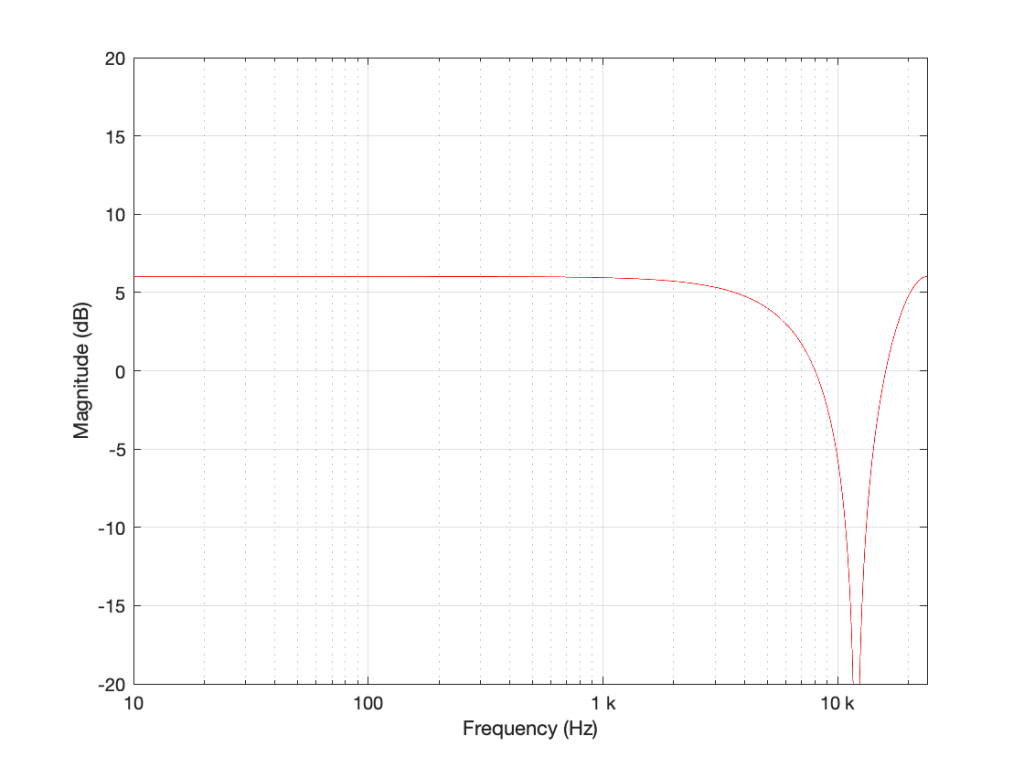Figure 4: The magnitude response of the filter shown in Figure 1 when the delay is set to 2 samples, the gain is set to 1 and Fs=48 kHz.

Again, if we reduce the gain, we reduce the effect of the filter, as can be seen in Figure 5.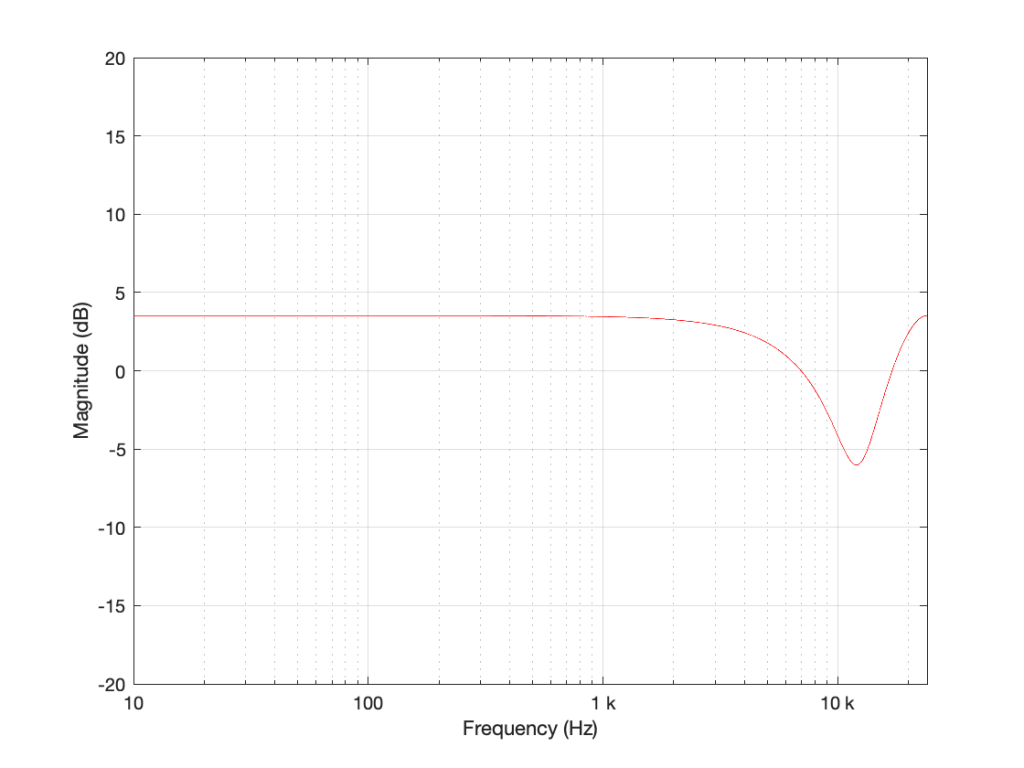Figure 5: The magnitude response of the filter shown in Figure 1 when the delay is set to 2 samples, the gain is set to 0.5 and Fs=48 kHz.

Now let’s make things a little more complicated. We can add another delay and another gain to get a little more control of things.

I’m not going to get very detailed about this – but if each of those delays is just one sample long, and we only play with the gains g1 and g2, we can start getting some nice control over the response of this filter. Below are some examples of the results we can get with just this filter, playing with the gains.

So, as you can see, all I need to do is to play with those two gains to get some nice control over the magnitude response.

Up to now, everything I’ve done is to add a delayed copy of the input to itself. This is what is known as a “feed-forward” design because (as you can see in Figures 1 and 6) I’m feeding the signal forwards in the flow to be added to itself. However, if there’s a “feed-forward”, it must be because we want to distinguish it from a “feed-back” design.

This is a filter where we delay the output (instead of the input) multiply that by a gain, and add it to the signal, as shown in Figure 11.

This feedback means that, if the gain is not equal to zero, once a signal gets into the input, the output will last forever. This is why this kind of filter is called an infinite impulse response filter (or IIR filter): because if an impulse (a short spike) gets into it, there will be a signal at the output until the end of time (theoretically, at least…).

And, yes… the output of a filter without a feedback loop will eventually stop, which means it’s a finite impulse response filter (or FIR). Stop the input signal, wait for the last delay to send its signal through, and the output stops.

## So what?

Most filters in most digital audio devices are built on a combination of these two types of designs. If you take Figure 6 and you combine it with an extended version of Figure 11 (with two delays instead of just one) you get Figure 12: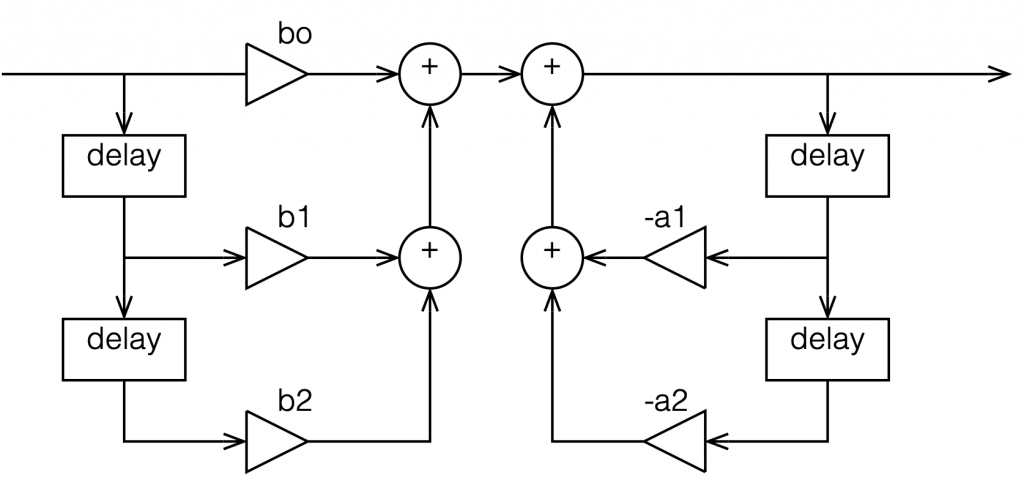Figure 12: An FIR filter with two delays and 3 gains combined with an IIR filter with two delays and 2 gains.

This combination of FIR and IIR filters is a powerful little tool that forms the heart of almost every digital audio filter in the world. (yes, there are exceptions, but they’re definitely exceptions…). There are different ways to implement it (for example, you could put the IIR before the FIR, or you could re-draw it to share the delays) but the result is the same.

This little tool is what we call a “biquadratic filter” or “biquad” for short. (The reason it’s called that is that the effect it has on the signal (its “transfer function”) can be mathematically expressed as the ratio of two quadratic equations – but I will not say anything more about that.) Whenever developers are building a new digital audio device like a loudspeaker or a pair of headphones or a car audio system, it’s common in the early meetings to hear someone ask “how many biquads will we need?” which is a way of asking “how much processing power and memory will we need?” (In the same way that I can measure prices in pizzas, or when I was a kid I would ask “how many more Sesame Streets until we’re there?”)

At this point, you may be asking why I’ve gone through all of this, since I haven’t said anything about high resolution audio. The reason is that, in the next posting, we’ll look at what’s going on inside that biquad when you use it to do filtering – and how that changes, not only with the filters you’re building, but how they relate to the sampling rate and the bit depth…

# “High-Res” Audio: Part 7: Conversion

Back in Part 5 of this series, I described an example of a pretty typical / normal signal flow for an audio signal that you’re playing from a streaming service to a “smart-ish” loudspeaker in your house. If you read through that list, you’ll see that I mentioned that the signal might be sampling-rate converted two times (once in your player, and once again in your loudspeaker or headphones).

Let me say something very clearly, before we go any further:

• There’s no guarantee that this is happening.
For example, many players don’t sampling-rate convert the signal if the device they’re sending the signal is compatible with the sampling rate of the signal. However, many players do sampling-rate convert the signal – and many devices (like DACs, for example) are not compatible with all sampling rates, so the player is forced to do something about it.
• Sampling rate conversion is not necessarily a bad thing.
There are many good sampling rate converters out there in the world. In fact, you can use a high-quality sampling rate converter to reduce problems with jitter coming in from an “upstream” device or transmission path.

However, sampling rate conversion is not necessarily a good thing either… so the more of them you have in your audio signal path, the better you want them to be. In an optimal case, the artefacts caused by the sampling rate converter will not be the “weakest link” in the audio chain.

However, this last statement is very easy to mis-interpret, as I alluded to in Part 6. The problem is that, if I say “I have a sampling rate converter with a THD+N of -100 dB relative to the signal level” this might look pretty good. However, if the signal and the SRC artefacts are in COMPLETELY different frequency bands, and you’re playing the signal out of a loudspeaker that can’t produce the signal (say, because it’s too low in frequency) then 100 dB might not be nearly good enough. In other words, it’s not a mere numbers-game… you have to know how to interpret the data…

## A what?

Maybe we should first back up a little and talk about what a sampling rate converter is. As you saw in Part 1, at its most basic level, LPCM digital audio is just a way of describing a signal by storing a long string of measurements that were made at a regular time interval. Each of those measurements is called a “sample” and the rate at which you measure the samples (per second) is called the “sampling rate”. A CD, for example, uses a standard sampling rate of 44,100 samples per second, or 44.1 kHz. Other systems use other rates.

If you want to listen to a CD on a loudspeaker with built-in digital processing, and the loudspeaker happens to have an internal sampling rate that is NOT 44.1 kHz (let’s say that it’s 48 kHz), then you need to somehow convert the sampling rate from 44.1 kHz to 48 kHz to get things to work properly. (This is a little like having a gearbox in a car – your engine does not turn at the same speed as your wheels – you put gears in-between to convert the rotational speed of the engine to the rotational speed of the wheels.)

One sneaky way to do this is to use an analogue connection – you convert the 44.1 kHz digital signal to an analogue one using a DAC, and then re-sample the analogue signal using an ADC running at 48 kHz. This is simple, and (if you choose your DAC and ADC properly) potentially a really good solution. In the “old days” (up to the 1990s) before digital SRCs became really good, this was the best way to do it (assuming you had access to some decent gear).

There are many ways to make a fully-digital SRC. For example:

Let’s say that you have an audio signal that’s been sampled at some sampling rate that we’ll call “Fs1” (for “Sampling Frequency 1”) , as is shown in Figure 1.

You then want to have the same signal, represented at a different sampling rate, which we’ll call Fs2. The old signal (in black) and the new sampling rate (the red dots and the gridlines) can be seen in Figure 2.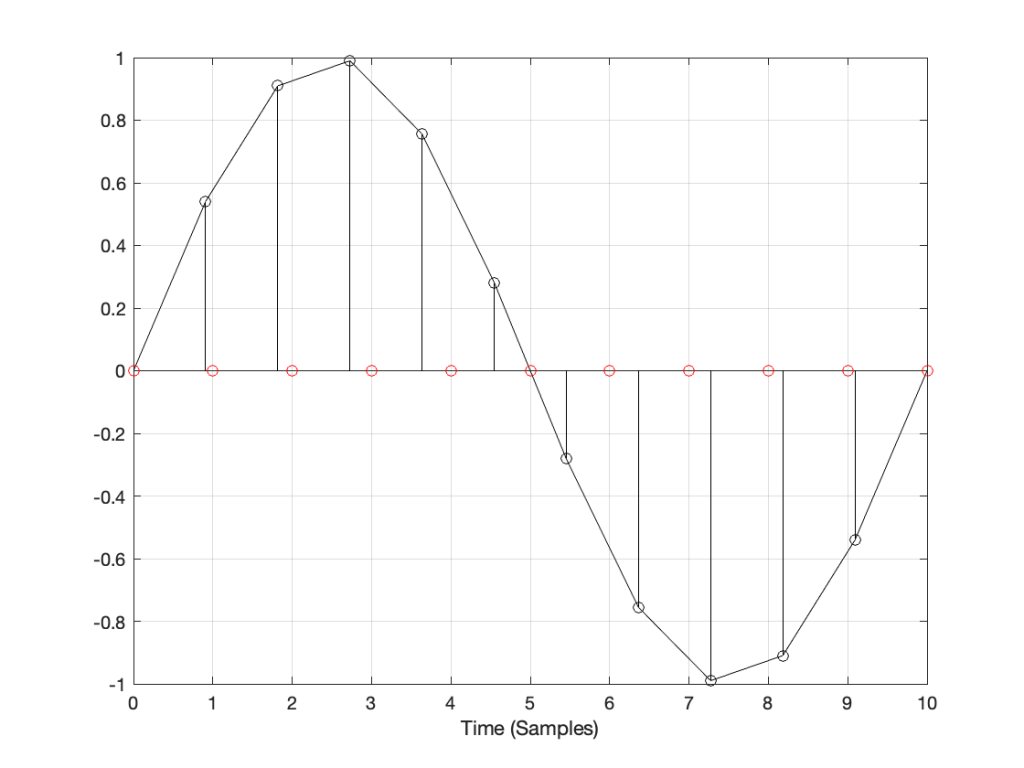Figure 2: The original signal at Fs1 and the new samples that we want to create (in red) at Fs2.

How do we do this? One way is to draw straight lines between the original samples, and calculate the values at the point on the line that corresponds with the time of the new samples. This is called “linear interpolation” (because it’s based on drawing straight lines between the original samples), and it’s shown in Figure 3.

A better way to do this is to use some fancy math to calculate where the signal would be after the reconstruction filter smoothed it back to the original (band-limited) input. There are different ways to do this (in other words, different mathematical strategies) that are outside the scope of this posting, however, I’ve shown an example of a piecewise cubic spline interpolation implementation in Figure 4, below.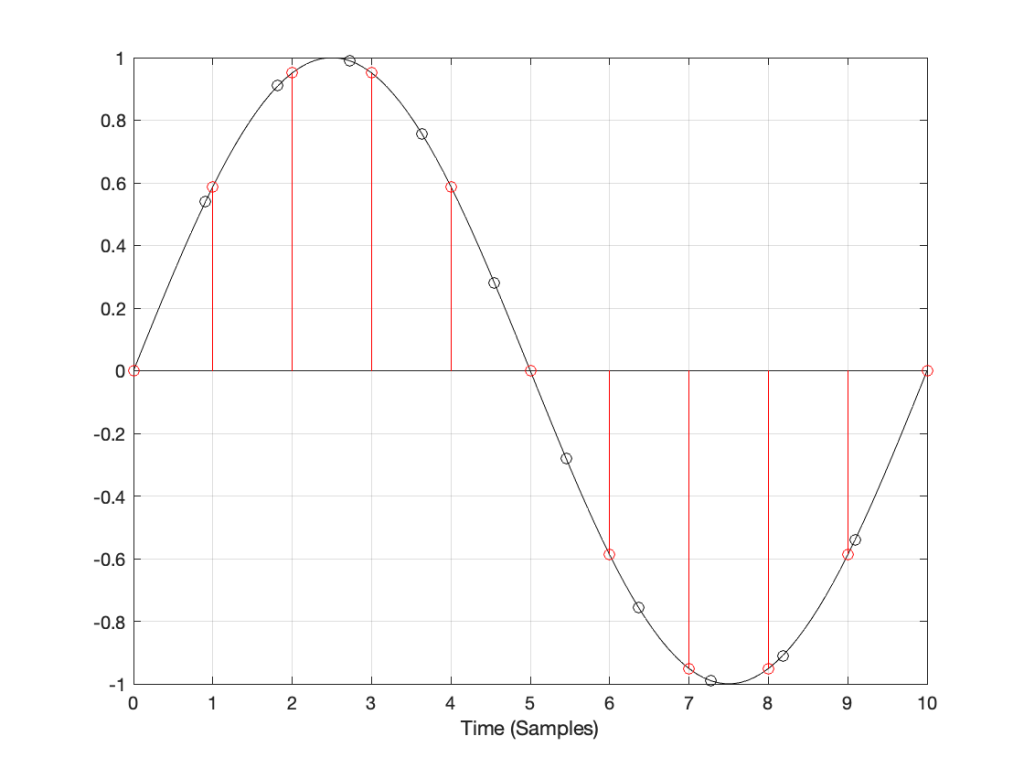Figure 4: An example of piecewise cubic spline interpolation for converting to the new sampling rate.

However, let’s say that:

• you’ve been given the job of building a sampling rate converter, but
• you think that the examples I gave above are way to complicated…

What do you do? One possibility is to look at the sample value that you want to output, find the closest sample (in time) in the original signal, and use that. This is a technique commonly called “nearest neighbour” for obvious reasons – and it’s one of the worst-performing SRC strategies you can use. An example of this is shown in Figure 5, below. Notice that the new values (the red circles) are identical to the closest original value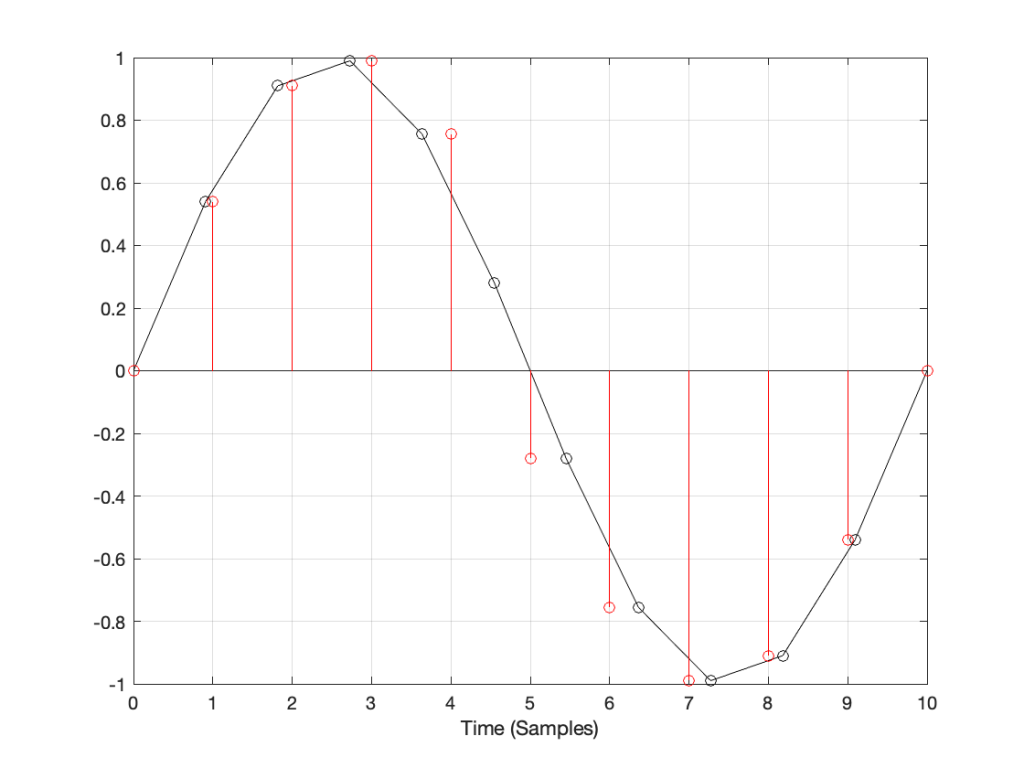Figure 5: An example of “nearest neighbour” interpolation for converting to the new sampling rate. Note that each new values in red is a copy of the closest value in black.

If we look at these two signals without the sample values, we’ll see some pretty nasty distortion in the time domain, as shown in Figure 6.

## So what?

The plots above show the results of good and bad SRCs in the time domain, but what does this look like in the frequency domain? Let’s take a couple of specific examples.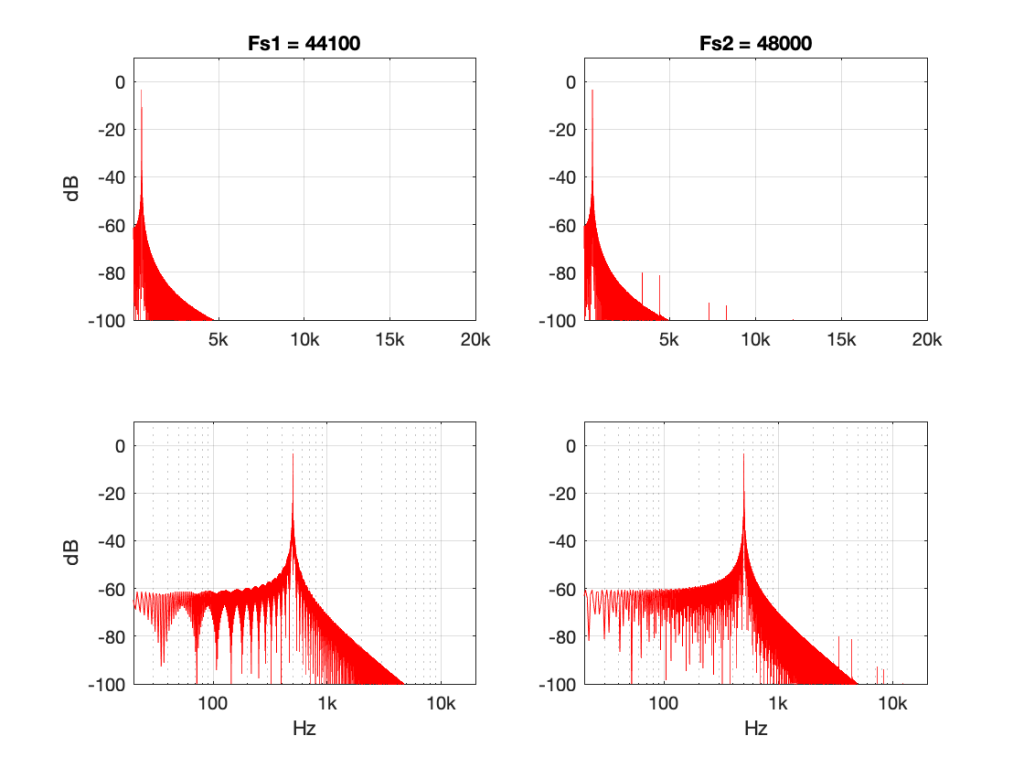Figure 7: 500 Hz sine tone at 0 dB FS, Linear interpolation from 44.1 kHz to 48 kHz.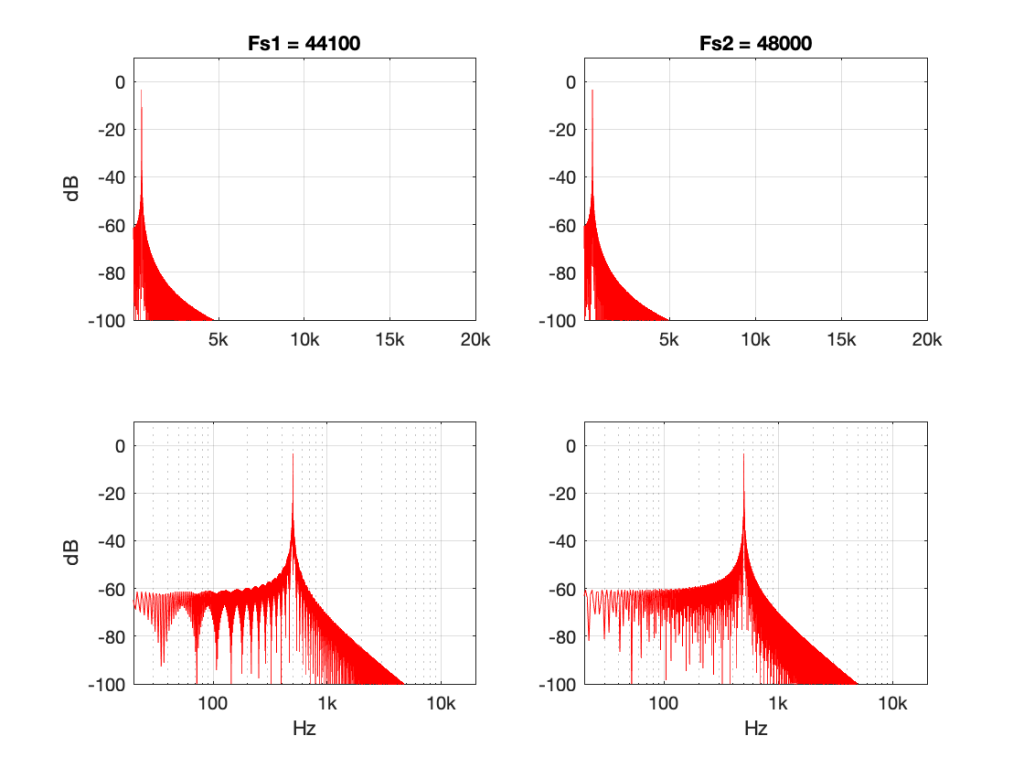Figure 8: 500 Hz sine tone at 0 dB FS, Piecewise cubic spline interpolation from 44.1 kHz to 48 kHz.

Figures 7 and 8 look almost identical. There are the windowing artefacts of the frequency analysis that I’m doing are larger than most of the artefacts caused by the interpolation implementations. However, you may notice a couple of spikes sticking up between 1 kHz and 10 kHz in Figure 7. These are the most obvious frequency-domain artefacts of the distortion caused by linear interpolation. Notice however, that those artefacts are about 80 dB down from the signal – so that’s pretty good for a cheap implementation.

However, let’s look at the same 500 Hz tone converted using the “nearest neighbour” strategy.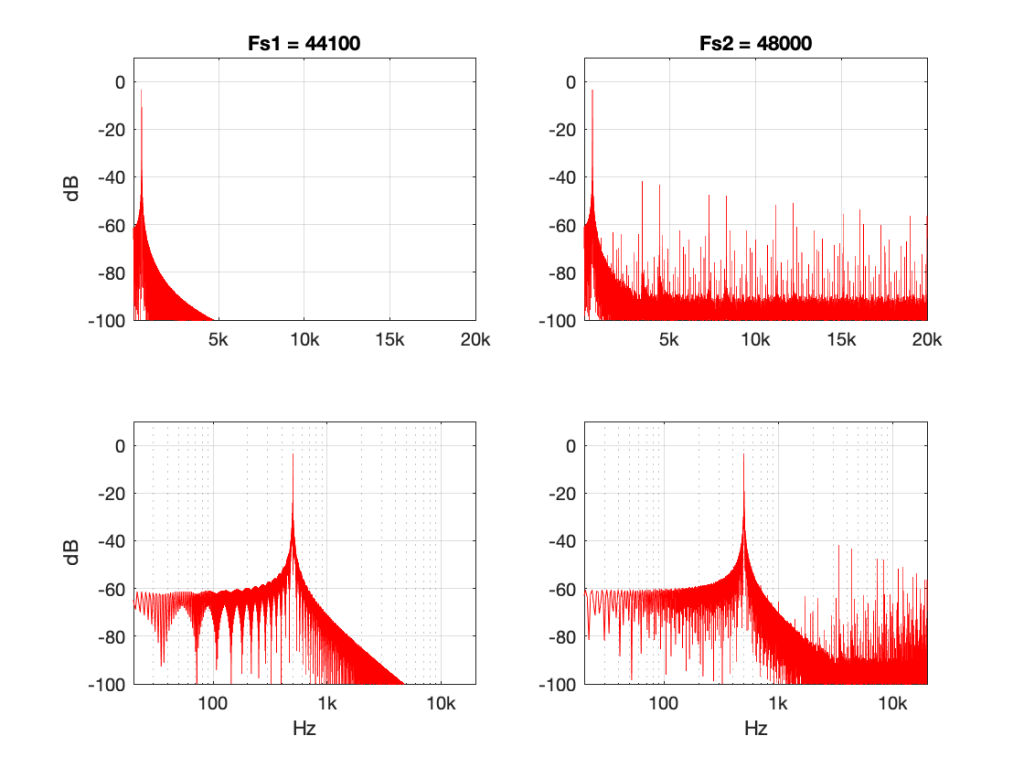Figure 9: 500 Hz sine tone at 0 dB FS, “nearest neighbour” interpolation from 44.1 kHz to 48 kHz.

Now you can see that things have really fallen apart The artefacts are almost up to 40 dB below the signal level, and they’re quite far away in frequency, so they’ll be easy to hear. Also remember that the artefacts that are generated here are inside the audio band, so they will not be eliminated later in the chain by a reconstruction filter in a DAC, for example. They’re there to stay.

There’s one more interesting thing to consider here. Let’s try the same nearest neighbour algorithm, converting between the same two sampling rates, but I’ll put in signals at different frequencies.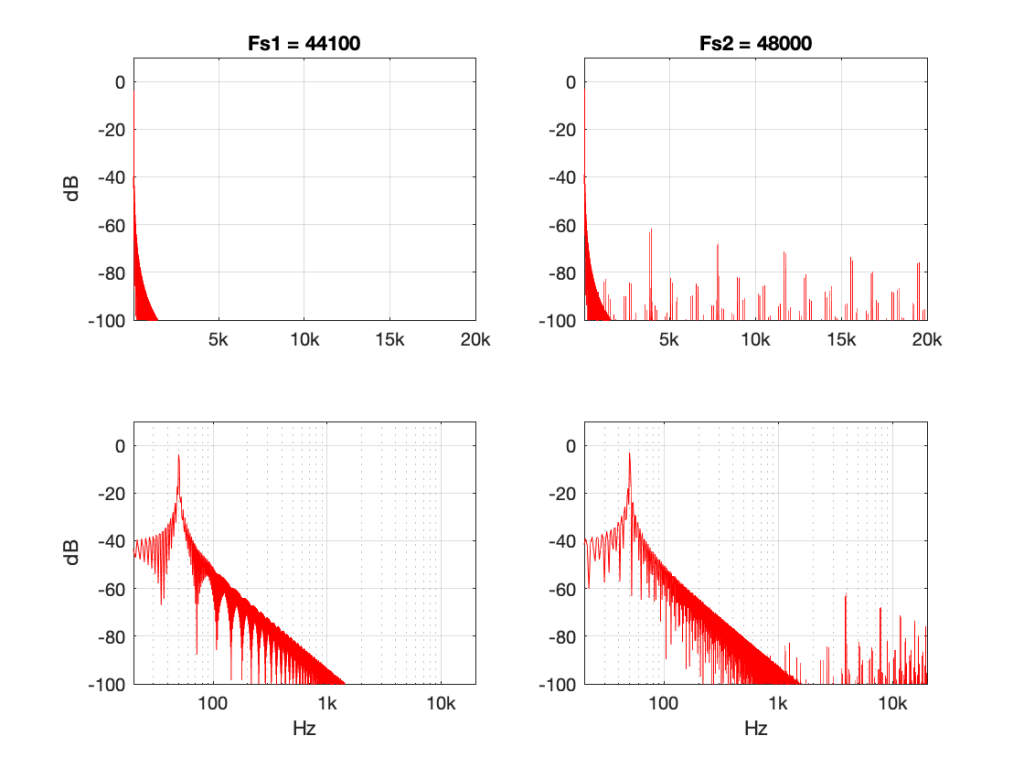Figure 10: 50 Hz sine tone at 0 dB FS, “nearest neighbour” interpolation from 44.1 kHz to 48 kHz.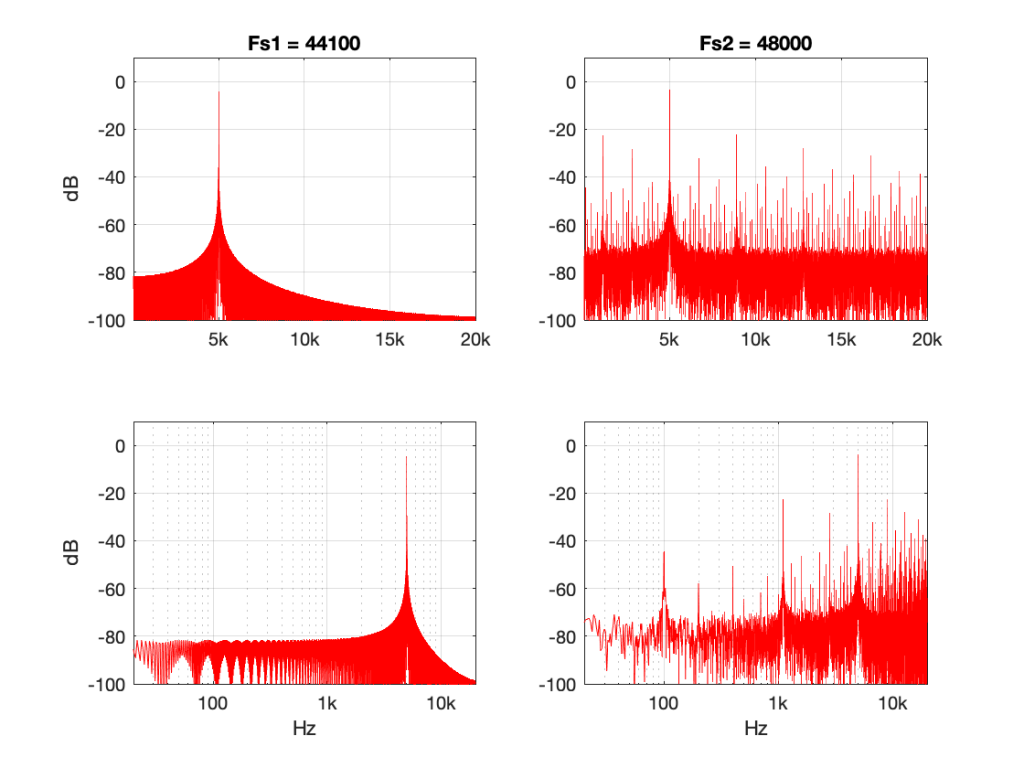Figure 11: 5 kHz sine tone at 0 dB FS, “nearest neighbour” interpolation from 44.1 kHz to 48 kHz.

Figure 10 shows the same system, but the input signal is a 50 Hz sine wave (instead of 500 Hz). Notice that the artefacts are now about 60 dB down (instead of 40 dB).

Figure 11 shows the same system again, but the input signal is a 5 kHz sine wave. Notice that the artefacts are now only about 20 dB down.

So, with this poor implementation of an SRC, the distortion-to-signal ratio is not only dependent on the algorithm itself, but the signal’s frequency content. Why is this?

Think back to the way the “nearest neighbour” strategy works. You’re simply copying-and-pasting the value of the nearest sample. However, the lower the frequency, the less change there is in the signal from sample to sample. So, as your signal’s frequency goes down (more accurately, as it gets further away from the sampling rate), the smaller the error that you create with this system. At 0 Hz, there would be no error, because all of the samples would have the same value.

So, (for example) if your job is to build the SRC in the first place, and you measure it with a 50 Hz tone, you’ll see that the artefacts are 60 dB below the signal and you’ll pat yourself on the back and go to lunch. Then, some weeks later, when the customer complaints start coming in about tweeter distortion, you’ll think it must be someone else’s fault… but it isn’t…

## Conclusion

What does this have to do with “High Resolution Audio”? Well, the problem is that most audio gear does not run at crazy-high sampling rates (this is not necessarily a bad thing), so if you play a high-res file, you’re probably sampling rate converting (this is not necessarily a bad thing).

However, if your gear does have a bad SRC in the signal flow (and, yes, this is not uncommon with modern audio gear) then you either need to

• play the signal with a different (e.g. not-high-res) sampling rate to find out if it’s better,
OR
OR
• at least check for a firmware update.

Note that first recommendation of the three: Because the quality of a sampling rate converter is very dependent upon the relationship between the input and the output sampling rates, it can happen that a “normal” resolution audio signal (say, at 44.1 kHz) will sound better on your particular equipment than a “high” resolution audio signal (say, at 192 kHz) because of this. Of course, the opposite could be true (say, because your gear is running at 48 kHz and it’s easier to get to that from 192 kHz (just multiply by 1/4) than it is to get there from 44.1 kHz (just multiply by 480/441…)

This doesn’t mean that “low-res” is better than “high-res” – it just means that your particular equipment deals with it better. (In the same way that purely from the point of view as a fuel, gasoline might have more energy per litre than diesel fuel, but it’s a terrible choice to put in the tank of a car that’s expecting diesel…)

# “High-Res” Audio: Part 6: Noise, noise, noise

In Part 2 of this series, I talked about the relationship between the noise floor of an LPCM signal and the number of bits used to encode it.

Assuming that the signal is correctly dithered using TPDF dither with a peak-to-peak amplitude of ±1 LSB, then this means that you can easily calculate the dynamic range of your system with a very simple equation:

Dynamic Range in dB = 6.02 * NumberOfBits – 3

(Note that the sampling rate is not part of this equation… That will be useful information later.)

Normally, we’re lazy and we say 6 times the number of bits -3 for the dither – but if you’re really lazy, you leave out the -3 as well.

So, this means that, in a 16-bit system, the noise floor is 93 dB below a sine wave at full scale (6 * 16 -3 = 93) and for a 24-bit system, the noise floor is 141 dB below a sine wave at full scale (you do the math as practice).

Also, we can generalise and say that “adding 1 bit halves the level of the noise floor” (because -6 dB is the same as multiplying by 0.5). However, this is only part of the story.

The noise that’s generated by dither has a “white” characteristic. This means that there is an equal probability of getting some energy per bandwidth (or some say “per Hertz”) over a period of time. This sounds a little complicated, so I’ll explain.

Noise is random. This means that you may or may not get energy at, say 1 kHz, in a given short measurement. However, if you measure white noise for long enough, you’ll eventually see that you got something in every frequency band. Also, you’ll see that, if you look back over the entire length of your measurement of white noise, you got the same amount of energy in the band from 100 Hz to 200 Hz as you did in the band from 1000 Hz to 1200 Hz and the band from 10,000 Hz to 10,200 Hz. (Each of those bandwidths is 200 Hz wide).

There are now two things to discuss:

1. This distribution of energy is not like the way we hear things. We don’t hear the distance between 100 Hz and 200 Hz as the same distance as going from 1,000 Hz to 1,200 Hz. We hear logarithmically, which means that we hear in multiples of frequency, not additions of bandwidth. So, to use 100 Hz – 200 Hz sounds like the same “distance” as 1,000 Hz to 2,000 Hz. This is why white noise sounds like it is “bright” – or it has emphasis on the high frequencies. If you have a system that has a flat response from 0 to 20,000 Hz, and you play white noise through it, you have the same amount of energy in the top octave (10 kHz to 20 kHz) as you do in all of the octaves below – which is why we hear this as “top-heavy”.
2. If you had two bands of white noise with equal levels, and let’s say that one ranges from 100 Hz to 200 Hz, and the other is 1000 Hz to 1200 Hz, then the output level of the two of them together will be 3 dB louder than the output level of either of them alone. This is because their powers add together instead of their amplitudes (because the two signals are unrelated to each other).

Let’s put all this (and one or two other things) together:

1. We know from a previous part in this series that an LPCM digital audio system cannot have signals higher than the Nyquist frequency – 1/2 the sampling rate.
2. TPDF dither is white noise at a total level that is (6.02 * NumberOfBits – 3) dB below full-scale.
3. If you add white noise signals with equal levels but different bandwidths, you get a 3 dB increase over the level of just one of them

This means that,

• if I have a 16-bit, TPDF dithered LPCM audio signal with a sampling rate of 48 kHz, it has a noise floor that is 93 dB below full scale, and that noise has a white characteristic with a bandwidth of 24 kHz (the Nyquist frequency). There will be no noise above that frequency coming out of the system.
• if I have a 16-bit, TPDF dithered LPCM audio signalwith a sampling rate of 192 kHz, it has a noise floor that is 93 dB below full scale, and that noise has a white characteristic with a bandwidth of 96 kHz (the Nyquist frequency). There will be no noise above that frequency coming out of the system.

So, the two systems have the same noise floor level overall, but with very different bandwidths… What does this mean?

Well, let’s start by looking at the level of the noise floor in the 48 kHz system (so the noise “only” extends to 24 kHz).

If I double the sampling rate (to 96 kHz), I double the bandwidth of the noise without changing its level, so this means that the portion of the noise that “lives” in the 0 Hz – 24 kHz region drops by 3 dB (because I’m ignoring the top half of the signal ranging from 24 kHz to 48 kHz in the 96 kHz system.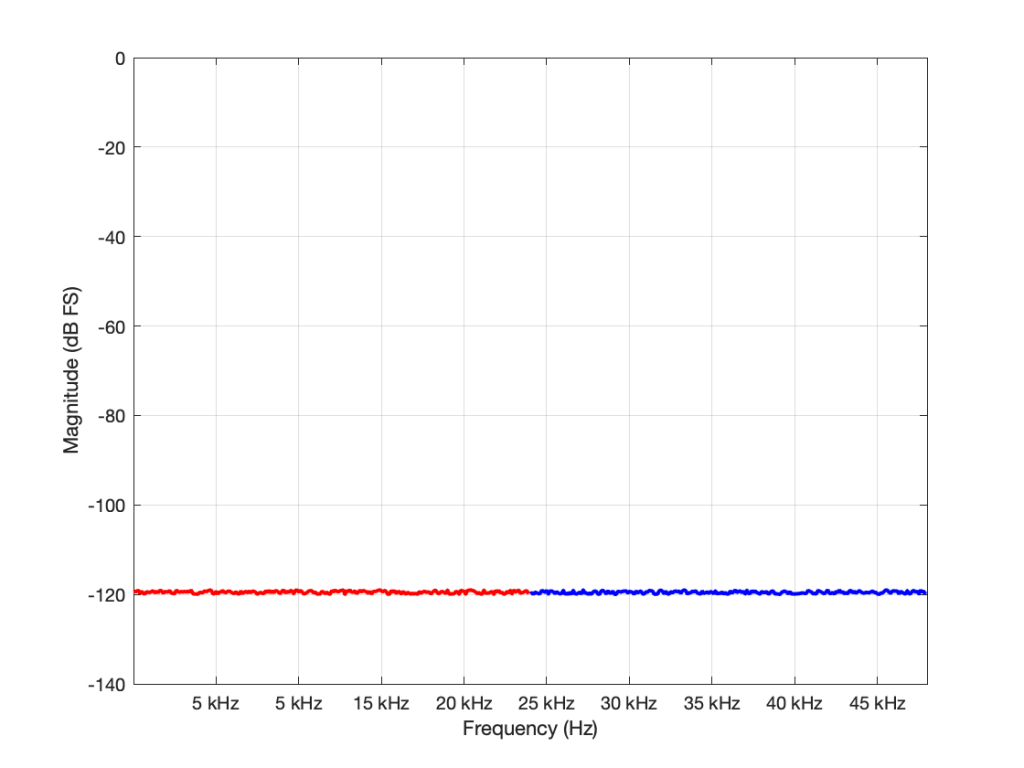Figure 1: The noise floor caused by TPDF dither in a 16-bit LPCM system with a sampling rate of 96 kHz. The total power of the noise drawn in red is the same as the total power of the noise drawn in blue. But notice that this is plotted on a linear scale for frequency.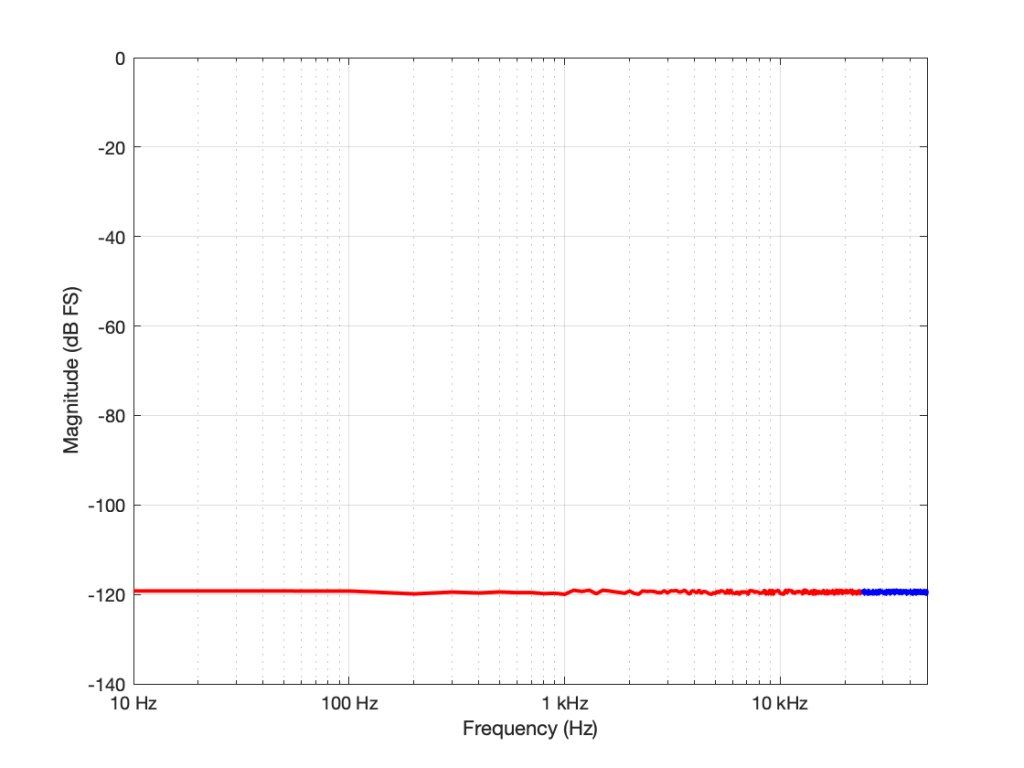Figure 1: Re-plotted on a logarithmic scale for the frequency. To us, the top octave is not so important, but to a measurement device, it’s half the signal…

If I had multiplied the original sampling rate by 4 (to 192 kHz) I multiply the bandwidth of the noise by 4 as well (to 96 kHz). This means that the overall level of the noise from 0 to 24 kHz is now 6 dB down from the original version.

In other words: if I multiply the sampling rate by two, but I don’t increase the bandwidth of the noise floor that I’m interested in (say I only care about 20 Hz – 20 kHz), then its level drops by 3 dB.

## So what?

Well, you could jump to the conclusion that this proves that higher sampling rates are better. However, that would be a bit (ha hah) premature. Consider that, if you want to drop the (band-limited) noise floor by 6 dB, you have to quadruple the sampling rate – and therefore quadrupling the data rate (and therefore the disc storage, the bandwidth of the transmission system, the error rate, and so on…) A 400% increase in the data is not insignificant.

OR, you could just add one more bit – going from 16 bits to 17 bits will give you the same result with a data increase of only 6.25% – a much smarter decision, no?

## The Real World

This little analysis above makes a basic (and possibly incorrect) assumption. The assumption is that, by quadrupling the sampling rate, all other components in the system will remain predictably identical. This may not be true. For example, many DACs (especially older ones) exhibit an increase in their own noise floor when you use them at a higher sampling rate. So, it could be that the benefit you get theoretically is negated by the detriment that you actually get. This is just one example of a flaw in the theory – but it’s a very typical one – especially if you’re building a product instead of just using one.

## P.S.

You may have looked at Figures 1 or 2 and are wondering why, if the noise floor is at -93 dB FS in a 16-bit system, I plotted it around -120 dB FS (give or take). The reason is related to the explanation I just gave above. I said in the captions that it’s from a 96 kHz system. This means that the noise extends to the Nyquist frequency at 48 kHz, and that total level is at -93 dB FS. We also know that, if I keep the noise the same, but half the bandwidth that I’m looking at, the level drops by 3 dB. Therefore I can either do math or I can make the following table:

If you look carefully at the figures, you’ll see that there’s a point every 100 Hz. (It’s most easily visible in the low-frequency range of Figure 2.) So, the level of the noise that I see on a magnitude response plot like this is not only dependent on the noise level itself, but the bandwidths of the divisions that I’ve used to slice it up. In my case, the bandwidth per “slice” is about 100 Hz, so the noise level of each of those little contributors is at about -120 dB FS. If I had used slices only 50 Hz wide, it would show up at -123 instead…

# “High-Res” Audio: Part 5 – Mirrors are bad

Let’s go back to something I said in the last post:

## Mistake #1

I just jumped to at least three conclusions (probably more) that are going to haunt me.

The first was that my “digital audio system” was something like the following:

As you can see there, I took an analogue audio signal, converted it to digital, and then converted it back to analogue. Maybe I transmitted it or stored it in the part that says “digital audio”.

However, the important, and very probably incorrect assumption here is that I did nothing to the signal. No volume control, no bass and treble adjustments… nothing.

If you consider that signal flow from the position of an end-consumer playing a digital recording, this was pretty easy to accomplish in the “old days” when we were all playing CDs. That’s because (in a theoretical, oversimplified world…)

• the output of the mixing/mastering console was analogue
• that analogue signal was converted to digital in the mastering studio
• the resulting bits were put on a disc
• you put that disc in your player which contained a DAC that converted the signal directly to analogue
• you then sent the signal to your “processing” (a.k.a. “volume control”, and maybe some bass and treble adjustment.).

So, that flowchart in Figure 1 was quite often true in 1985.

These days, things are probably VERY different… These days, the signal path probably looks something more like this (note that I’ve highlighted “alterations” or changes in the bits in the audio signal in red):

• The signal was converted from analogue to digital in the studio
(yes, I know… studios often work with digital mixers these days, but at least some of the signals within the mix were analogue to start – unless you are listening to music made exclusively with digital synthesizers)
• The resulting bits were saved on a file
• Depending on the record label, the audio signal was modified to include a “watermark” that can identify it later – in court, when you’ve been accused of theft.
• The file was transferred to a storage device (let’s say “hard drive”) in a large server farm renting out space to your streaming service
• The streaming service encodes the file
• If the streaming service does not offer an lossless option, then the file is converted to a lossy format like MP3, Ogg Vorbis, AAC, or something else.
• If the streaming service offers a lossless option, then the file is compressed using a format like FLAC or ALAC (This is not an alteration, since, with a lossless compression system, you don’t lose anything)
(it might look like an audio player – but that means it’s just a computer that you can’t use to check your social media profile)
• You press play, and the signal is decoded (either from the lossy CODEC or the compression format) back to LPCM. (Still not an alteration. If it’s a lossy CODEC, then the alteration has already happened.)
• That LPCM signal might be sample-rate converted
• The streaming service’s player might do some processing like dynamic range compression or gain changes if you’ve asked it to make all the songs have the same level.
• All of the user-controlled “processing” like volume controls, bass, and treble, are done to the digital signal.
• The signal is sent to the loudspeaker or headphones
• If you’re sending the signal wirelessly to a loudspeaker or headphones, then the signal is probably re-encoded as a lossy CODEC like AAC, aptX, or SBC.
(Yes, there are exceptions with wireless loudspeakers, but they are exceptions.)
• If you’re sending the signal as a digital signal over a wire (like S/PDIF or USB), the you get a bit-for-bit copy at the input of the loudspeaker or headphones.
• The loudspeakers or headphones might sample-rate convert the signal
• The sound is (finally) converted to analogue – either one stream per channel (e.g. “left”) or one stream per loudspeaker driver (e.g. “tweeter”) depending on the product.

So, as you can see in that rather long and complicated list (it looks complicated, but I’ve actually simplified it a little, believe it or not), there’s not much relation to the system you had in 1985.

Let’s take just one of those blocks and see what happens if things go horribly wrong. I’ll take the “volume control” block and add some distortion to see the result with two LPCM systems that have two different sampling rates, one running at 48 kHz and the other at 194 kHz – four times the rate. Both systems are running at 24 bits, with TPDF dither (I won’t explain what that means here). I’ll start by making a 10 kHz tone, and sending it through the system without any intentional distortion. If we look at those two signals in the time domain, they’ll look like this: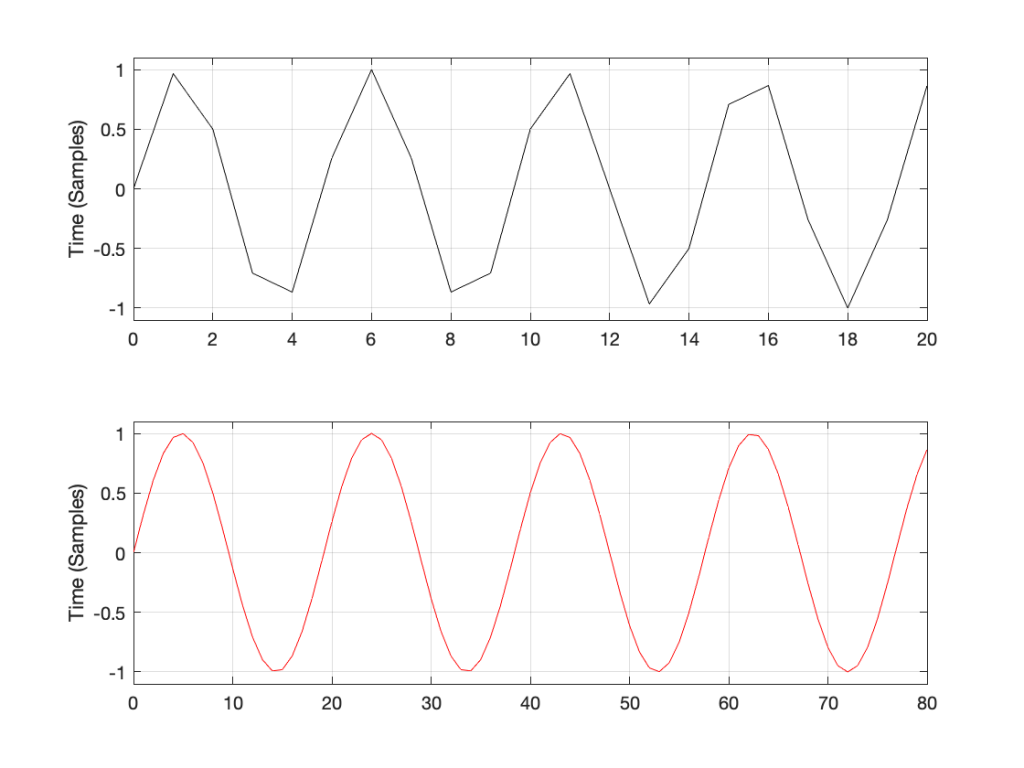Figure 1: Two 10 kHz tones. The black one is in a 48 kHz, 24 bit LPCM system. The red one is in a 192 kHz, 24 bit LPCM system.

The sine tone in the 48 kHz system may look less like a sine tone than the one in the 192 kHz system, however, in this case, appearances are deceiving. The reconstruction filter in the DAC will filter out all the high frequencies that are necessary to reproduce those corners that you see here, so the resulting output will be a sine wave. Trust me.

If we look at the magnitude responses of these two signals, they look like Figure 2, below.

You may be wondering about the “skirts” on either side of the 10 kHz spikes. These are not really in the signal, they’re a side-effect (ha ha) of the windowing process used in the DFT (aka FFT). I will not explain this here – but I did a long series of articles on windowing effects with DFTs, so you can search for it if you’re interested in learning more about this.

If you’re attentive, you’ll notice that both plots extend up to 96 kHz. That’s because the 192 kHz system on the bottom has a Nyquist frequency of 96 kHz, and I want both plots to be on the same scale for reasons that will be obvious soon.

Now I want to make some distortion. In order to make things obvious, I’m going to make a LOT of distortion. I’ve made the sine wave try to have an amplitude that is 10 times higher than my two systems will allow. In other words, my amplitude should be +/- 10, but the signal clips at +/- 1, resulting in something looking very much like a square wave, as shown in Figure 3.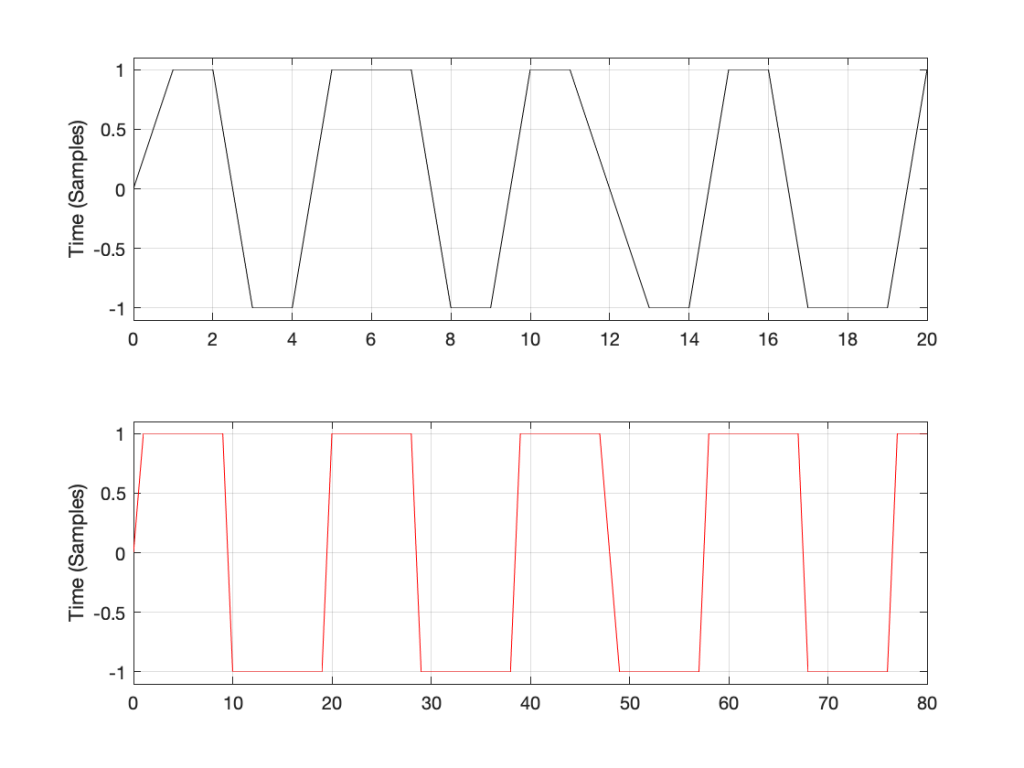Figure 3: Distorted 10 kHz sine waves. The black one is in a 48 kHz, 24 bit LPCM system. The red one is in a 192 kHz, 24 bit LPCM system.

You may already know that if you want to make a square wave by building it up using its constituent harmonics, you need to have the fundamental (which we’ll call Fc. In our case, Fc = 10 kHz) with an amplitude that we’ll say is “A”, you then add the

• 3rd harmonic (3 times Fc, so 30 kHz in our case) with an amplitude of A/3.
• 5th harmonic (5 Fc = 50 kHz) with an amplitude of A/5
• 7 Fc at A/7
• and so on up to infinity

Let’s look at the magnitude responses of the two signals above to see if that’s true.

If we look at the bottom plot first (running at 192 kHz and with a Nyquist limit of 96 kHz) the 10 kHz tone is still there. We can also see the harmonics at 30 kHz, 50 kHz, 70 kHz, and 90 kHz in amongst the mess of other spikes we’ll get to those soon…)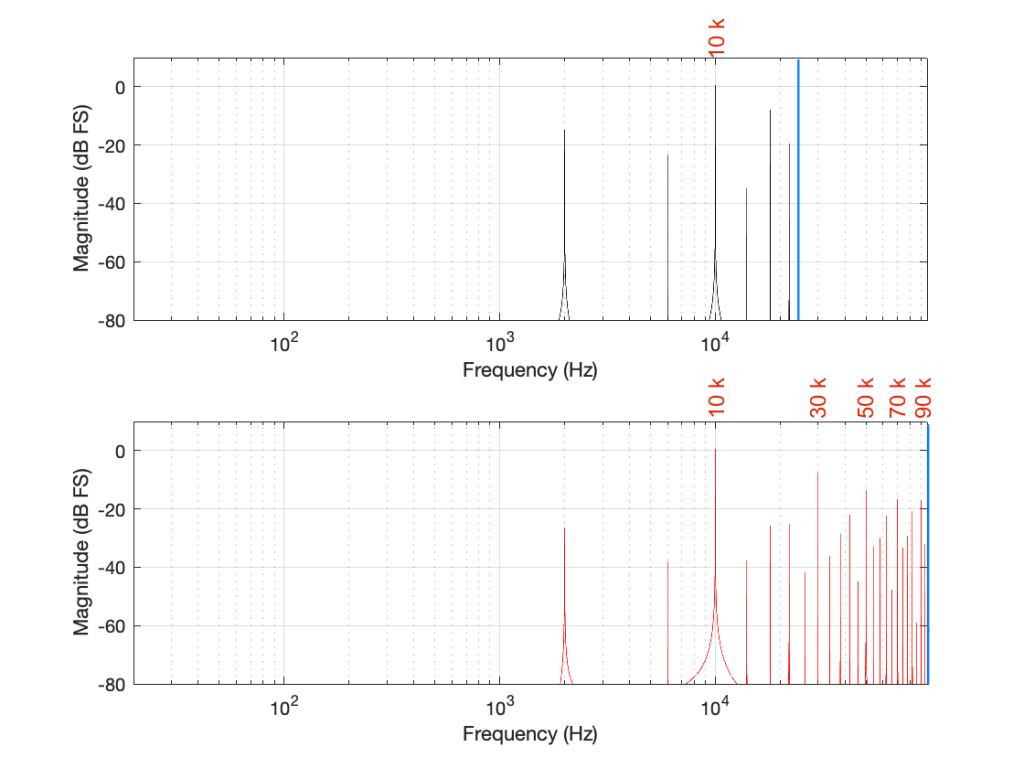Figure 5. Some labels applied to Figure 4 for clarity, showing the harmonics of the square waves that are captured by the two systems

Looking at the top plot (running at 48 kHz and with a Nyquist limit of 24 kHz), we see the 10 kHz tone, but the 30 kHz harmonic is not there – because it can’t be. Signals can’t exist in our system above the Nyquist limit. So, what happens? Think back to the images of the rotating wheel in Part 3. When the wheel was turning more than 1/2 a turn per frame of the movie, it appears to be going backwards at a different speed that can be calculated by subtracting the actual rotation from 180º (half-a-turn).

The same is true when, inside a digital audio signal flow, we try to make a signal that’s higher than Nyquist. The energy exists in there – it just “folds” to another frequency – its “alias”.

We can look at this generally using Figure 6.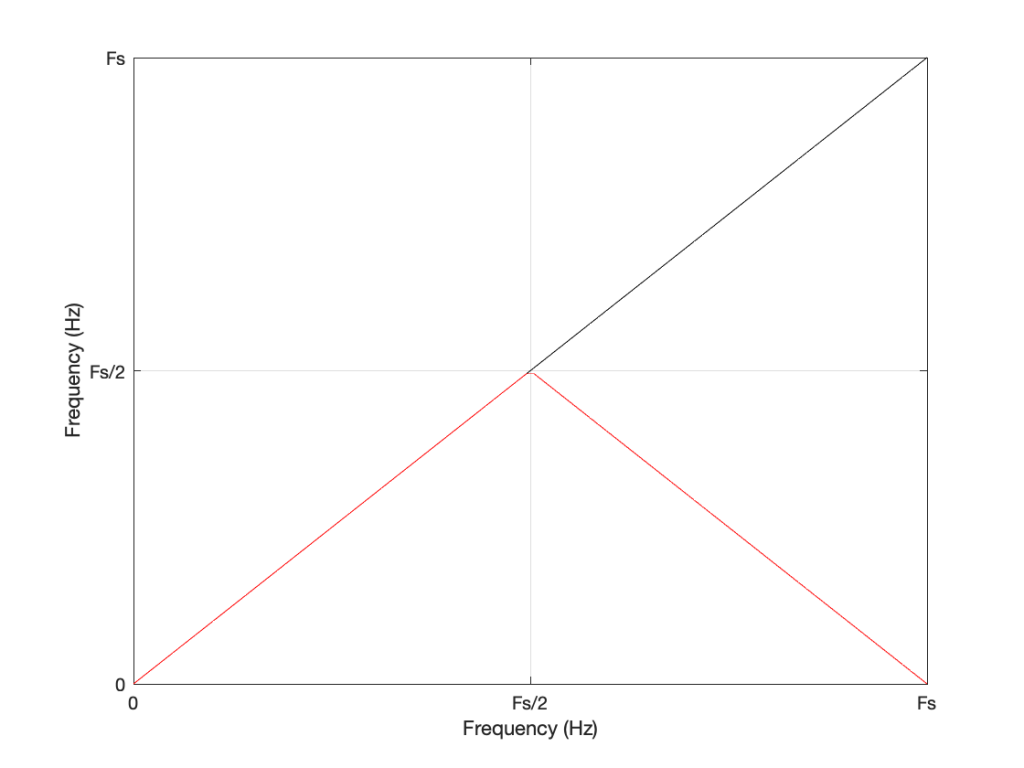Figure 6: A general plot of aliasing, showing the intended frequency in black and the actual output frequency in red.

Looking at Figure 6: If we make a sine tone that sweeps upward from 0 Hz to the Nyquist frequency at Fs/2 (half the sampling rate or sampling frequency) then the output is the same as the input. However, when the intended frequency goes above Fs/2, the actual frequency that comes out is Fs/2 minus the intended frequency. This creates a “mirror” effect.

If the intended frequency keeps going up above Fs, then the mirroring happens again, and again, and again… This is illustrated in Figure 7.

This plot is shown with linear scales for both the X- and Y-axes to make it easy to understand. If the axes in Figure 7 were scaled to a logarithmic scaling instead (which is how “Frequency Response” are normally shown, since this corresponds to how we hear frequency differences), then it would look like Figure 8.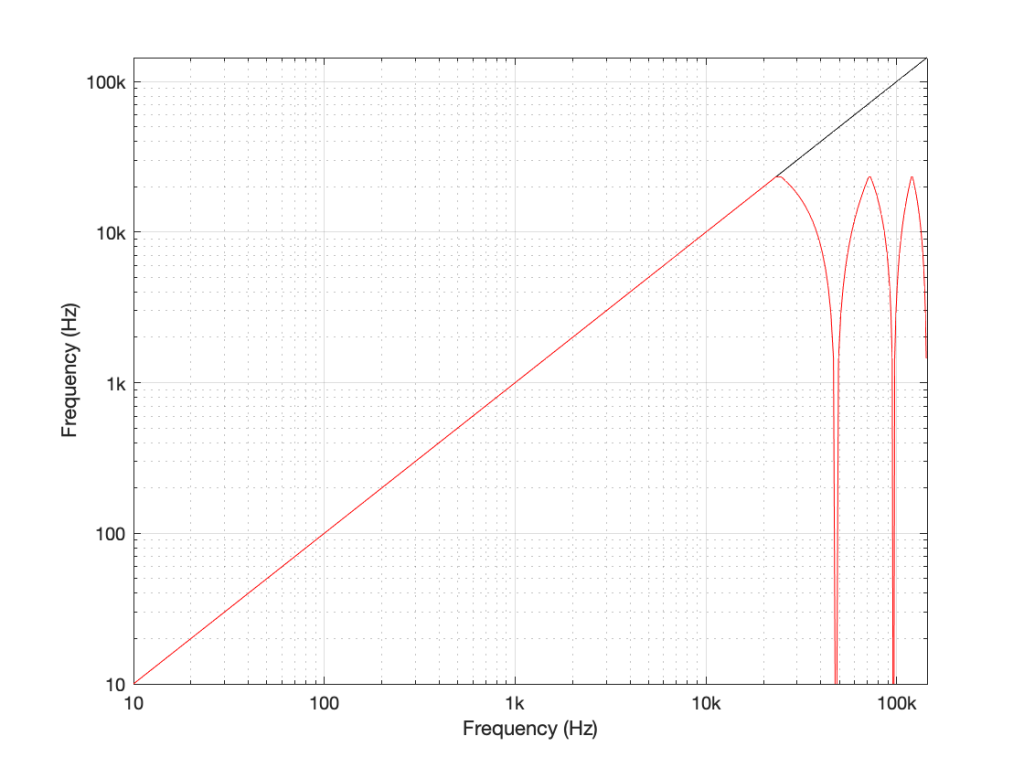Figure 8: The same information shown in Figure 7, plotted on a logarithmic scale instead. Note that this example is for a system running at 48 kHz (therefore with a Nyquist frequency of 24 kHz), and an intended input frequency (in black) going up to 3 times 48 kHz = 144 kHz.

Coming back to our missing 30 kHz harmonic in the 48 kHz LPCM system: Since 30 kHz is above the Nyquist limit of 24 kHz in that system, it mirrors down to 24 kHz – (30 kHz – 24 kHz) = 18 kHz. The 50 kHz harmonic shows up as an alias at 2 kHz. (follow the red line in Figure 7: A harmonic on the black line at 48 kHz would actually be at 0 Hz on the red line. Then, going 2000 Hz up to 50 kHz would bring the red line up to 2 kHz.)

Similarly, the 110 kHz harmonic in the 192 kHz system will produce an alias at 96 kHz – (110 kHz – 96 kHz) = 82 kHz.

If I then label the first set of aliases in the two systems, we get Figure 9.

Now we have to stop for a while and think about what’s happened.

We had a digital signal that was originally “valid” – meaning that it did not contain any information above the Nyquist frequency, so nothing was aliasing. We then did something to the signal that distorted it inside the digital audio path. This produced harmonics in both cases, however, some of the harmonics that were produced are harmonically related to the original signal (just as they ought to be) and others are not (because they’re aliases of frequency content that cannot be reproduced by the system.

What we have to remember is that, once this happens, that frequency content is all there, in the signal, below the Nyquist frequency. This means that, when we finally send the signal out of the DAC, the low-pass filtering performed by the reconstruction filter will not take care of this. It’s all part of the signal.

So, the question is: which of these two systems will “sound better” (whatever that means)? (I know, I know, I’m asking “which of these two distortions would you prefer?” which is a bit of a weird question…)

This can be answered in two ways that are inter-related.

The first is to ask “how much of the artefact that we’ve generated is harmonically related to the signal (the original sine tone)?” As we can see in Figure 5, the higher the sampling rate, the more artefacts (harmonics) will be preserved at their original intended frequencies. There’s no question that harmonics that are harmonically related to the fundamental will sound “better” than tones that appear to have no frequency relationship to the fundamental. (If I were using a siren instead of a constant sine tone, then aliased harmonics are equally likely to be going down or up when the fundamental frequency goes up… This sounds weird.)

The second is to look at the levels of the enharmonic artefacts (the ones that are not harmonically related to the fundamental). For example, both the 48 kHz and the 192 kHz system have an aliased artefact at 2 kHz, however, its level in the 48 kHz system is 15 dB below the fundamental whereas, in the 192 kHz system, it’s more than 26 dB below. This is because the 6 kHz artefact in the 48 kHz system is an alias of the 30 kHz harmonic, whereas, in the 192 kHz system, it’s an alias of the 190 kHz harmonic, which is much lower in level.

As I said, these two points are inter-related (you might even consider them to be the same point) however, they can be generalised as follows:

The higher the sampling rate, the more the artefacts caused by distortion generated within the system are harmonically related to the signal.

In other words, it gives a manufacturer more “space” to screw things up before they sound bad. The title of this posting is “Mirrors are bad” but maybe it should be “Mirrors are better when they’re further away” instead.

Of course, the distortion that’s actually generated by processing inside a digital audio system (hopefully) won’t be anything like the clipping that I did to the signal. On the other hand, I’ve measured some systems that exhibit exactly this kind of behaviour. I talked about this in another series about Typical Problems in Digital Audio: Aliasing where I showed this measurement of a real device: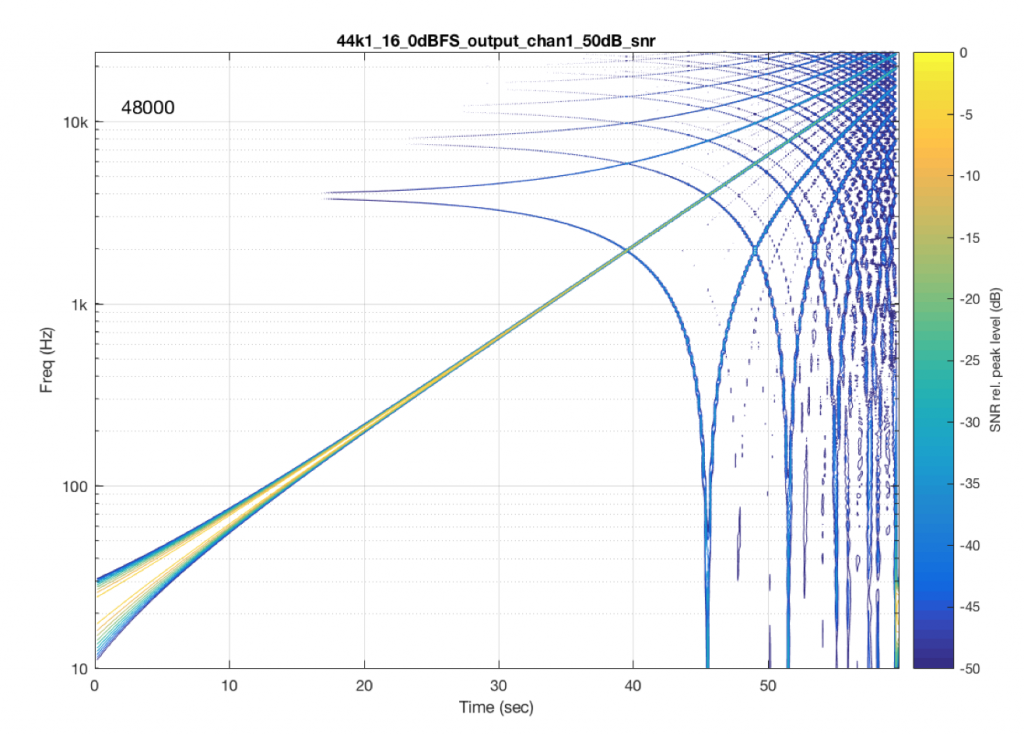Figure 10: A measurement of a real device showing some kind of distortion and aliased artefacts of a swept sine tone. Half of the aliasing is immediately recognizable as going downwards when the tone is going upwards.

However, I’m not here to talk about what you can or can’t hear – that is dependent on too many variables to make it worth even starting to talk about. The point of this series is not to prove that something is better or worse than something else. It’s only to show the advantages and disadvantages of the options so that you can make an informed choice that best suits your requirements.

On to Part 6

# Turn it down half-way…

#81 in a series of articles about the technology behind Bang & Olufsen loudspeakers

Bertrand Russell once said, “In all affairs it’s a healthy thing now and then to hang a question mark on the things you have long taken for granted.”

This article is a discussion, both philosophical and technical about what a volume control is, and what can be expected of it. This seems like a rather banal topic, but I find it surprising how often I’m required to explain it.

## Basic concepts

We need to start at the very beginning, so here goes:

### Volume control and gain

1. An audio signal is (at least in a digital audio world) just a long list of numbers for each audio channel.
2. The level of the audio signal can be changed by multiplying it by a number (called the gain).
1. If you multiply by a value larger than 1, the audio signal gets louder.
2. If you multiply by a number between 0 and 1, the audio signal gets quieter.
3. If you multiply by zero, you mute the audio signal.
3. Therefore, at its simplest core, a volume control implemented in a digital audio system is a multiplication by a gain. You turn up the volume, the gain value increases, and the audio is multiplied by a bigger number producing a bigger result.

That’s the first thing. Now we move on to how we perceive things…

### Perception of Level

Speaking very generally, our senses (that we use to perceive the world around us) scale logarithmically instead of linearly. What does this mean? Let’s take an example:

Let’s say that you have $100 in your bank account. If I then told you that you’d won$100, you’d probably be pretty excited about it.

However, if you have $1,000,000 in your bank account, and I told you that you’re won$100, you probably wouldn’t even bother to collect your prize.

This can be seen as strange; the second $100 prize is not less money than the first$100 prize. However, it’s perceived to be very different.

If, instead of being $100, the cash prize were “equal to whatever you have in your bank account” – so the person with$100 gets $100 and the person with$1,000,000 gets \$1,000,000, then they would both be equally excited.

The way we perceive audio signals is similar. Let’s say that you are listening to a song by Metallica at some level, and I ask you to turn it down, and you do. Then I ask you to turn it down by the same amount again, and you do. Then I ask you to turn it down by the same amount again, and you do… If I were to measure what just happened to the gain value, what would I find?

Well, let’s say that, the first time, you dropped the gain to 70% of the original level, so (for example) you went from multiplying the audio signal by 1 to multiplying the audio signal by 0.7 (a reduction of 0.3, if we were subtracting, which we’re not). The second time, you would drop by the same amount – which is 70% of that – so from 0.7 to 0.49 (notice that you did not subtract 0.3 to get to 0.4). The third time, you would drop from 0.49 to 0.343. (not subtracting 0.3 from 0.4 to get to 0.1).

In other words, each time you change the volume level by the “same amount”, you’re doing a multiplication in your head (although you don’t know it) – in this example, by 0.7. The important thing to note here is that you are NOT subtracting 0.3 from the gain in each of the above steps – you’re multiplying by 0.7 each time.

What happens if I were to express the above as percentages? Then our volume steps (and some additional ones) would look like this:

100%
70%
49%
34%
24%
17%
12%
8%

Notice that there is a different “distance” between each of those steps if we’re looking at it linearly (if we’re just subtracting adjacent values to find the difference between them). However, each of those steps is a reduction to 70% of the previous value.

This is a case where the numbers (as I’ve expressed them there) don’t match our experience. We hear each reduction in level as the same as the other steps, but they don’t look like they’re the same step size when we write them all down the way I’ve done above. (In other words, the numerical “distance” between 100 and 70 is not the same as the numerical “distance” between 49 and 34, but these steps would sound like the same difference in audio level.)

SIDEBAR: This is very similar / identical to the way we hear and express frequency changes. For example, the figure below shows a musical staff. The red brackets on the left show 3 spacings of one octave each; the distance between each of the marked frequencies sound the same to us. However, as you can see by the frequency indications, each of those octaves has a very different “width” in terms of frequency. Seen another way, the distance in Hertz in the octave from 440 Hz to 880 Hz is equal to the distance from 440 Hz all the way down to 0 Hz (both have a width of 440 Hz). However, to us, these sound like very different intervals.

SIDEBAR to the SIDEBAR: This also means that the distance in Hertz covered by the top octave on a piano is larger than the the distance covered by all of the other keys.

SIDEBAR to the SIDEBAR to the SIDEBAR: This also means that changing your sampling rate from 48 kHz to 96 kHz doubles your bandwidth, but only gives you an extra octave. However, this is not an argument against high-resolution audio, since the frequency range of the output is a small part of the list of pro’s and con’s.)

This is why people who deal with audio don’t use percent – ever. Instead, we use an extra bit of math that uses an evil concept called a logarithm to help to make things make more sense.

### What is a logarithm?

If I say the following, you should not raise your eyebrows:

2*3 = 6, therefore 6/2 = 3 and 6/3 = 2

In other words, division is just multiplication done backwards. This can be generalised to the following:

if a*b=c, then c/a=b and c/b=a

Logarithms are similar; they’re just exponents done backwards. For example:

102 = 100, therefore Log10(100) = 2

and generally:

AB=C, therefore LogA(C) = B

### Why use a logarithm?

The nice thing about logarithms is that they are a convenient way for a mathematician to do addition instead of multiplication.

For example, if I have the following sequence of numbers:

2, 4, 8, 16, 32, 64, and so on…

It’s easy to see that I’m just multiplying by 2 to get the next number.

What if I were to express the number sequence above as a series of exponents? Then it would look like this:

21, 22, 23, 24, 25, 26

Not useful yet…

What if I asked you to multiply two numbers in that sequence? Say, for example, 1024 * 8192. This would take some work (or at least some scrambling, looking for the calculator app on your phone…). However, it helps to know that this is the same as asking you to multiply 210 * 213 – to which the answer is 223. Notice that 23 is merely 10+13. So, I’ve used exponents to convert the problem from multiplication (1024*8192) to addition (210 * 213 = 2(10+13)).

How did I find out that 8192 = 213? By using a logarithm : Log2(8192) = 13.

In the old days, you would have been given a book of logarithmic tables in school, which was a way of looking up the logarithm of 8192. (Actually, those books were in base 10 and not base 2, so you would have found out that Log10(8192) = 3.9013, which would have made this discussion more confusing…) Nowadays, you can use an antique device called a “calculator” – a simulacrum of which is probably on a device you call a “phone” but is rarely used as such.

I will leave it to the reader to figure out why this quality of logarithms (that they convert multiplication into addition) is why slide rules work.

### So what?

Let’s go back to the problem: We want to make a volume slider (or knob) where an equal distance (or rotation) corresponds to an equal change in level. Let’s do a simple one that has 10 steps. Coming down from “maximum” (which we’ll say is a gain of 1 or 100%), it could look like these:

The plot above shows four different options for our volume controller. Starting at the maximum (volume step 10) and working downwards to the left, each one drops by the same perceived amount per step. The Black plot shows a drop of 90% per step, the red plot shows a drop of 70% per step (which matches the list of values I put above), Blue is 50% per step, and green is 30% per step.

As you can see, these lines are curved. As you can also see, as you get lower and lower, they get to the point where it gets harder to see the value (for example, the green curve looks like it has the same gain value for Volume steps 1 through 4).

However, we can view this a different way. If we change the scale of our graph’s Y-Axis to a logarithmic one instead of a linear one, the exact same information will look like this:

Notice now that the Y-axis has an equal distance upwards every time the gain multiplies by 10 (the same way the music staff had the same distance every time we multiplied the frequency by 2). By doing this, we now see our gain curves as straight lines instead of curved ones. This makes it easy to read the values both when they’re really small and when they’re (comparatively) big (those first 4 steps on the green curve don’t look the same on that plot).

So, one way to view the values for our Volume controller is to calculate the gains, and then plot them on a logarithmic graph. The other way is to build the logarithm into the gain itself, which is what we do. Instead of reading out gain values in percent, we use Bels (named after Alexander Graham Bell). However, since a Bel is a big step, we we use tenths of a Bel or “decibels” instead. (… In the same way that I tell people that my house is 4,000 km, and not 4,000,000 m from my Mom’s house because a metre is too small a division for a big distance. I also buy 0.5 mm pencil leads – not 0.0005 m pencil leads. There are many times when the basic unit of measurement is not the right scale for the thing you’re talking about.)

In order to convert our gain value (say, of 0.7) to decibels, we do the following equation:

20 * Log10(gain) = Gain in dB

So, we would say

20 * Log10(0.7) = -3.01 dB

I won’t explain why we say 20 * the logarithm, since this is (only a little) complicated.

I will explain why it’s small-d and capital-B when you write “dB”. The small-d is the convention for “deci-“, so 1 decimetre is 1 dm. The capital-B is there because the Bel is named after Alexander Graham Bell. This is similar to the reason we capitalize Hz, V, A, and so on…

So, if you know the linear gain value, you can calculate the equivalent in decibels. If I do this for all of the values in the plots above, it will look like this:

Notice that, on first glance, this looks exactly like the plot in the previous figure (with the logarithmic Y-Axis), however, the Y-Axis on this plot is linear (counting from -100 to 0 in equal distances per step) because the logarithmic scaling is already “built into” the values that we’re plotting.

For example, if we re-do the list of gains above (with a little rounding), it becomes

100% = 0 dB
70% = -3 dB
49% = -6 dB
34% = -9 dB
24% = -12 dB
17% = -15 dB
12% = -18 dB
8% = -21 dB

Notice coming down that list that each time we multiplied the linear gain by 0.7, we just subtracted 3 from the decibel value, because, as we see in the equation above, these mean the same thing.

This means that we can make a volume control – whether it’s a slider or a rotating knob – where the amount that you move or turn it corresponds to the change in level. In other words, if you move the slider by 1 cm or rotate the knob by 10º – NO MATTER WHERE YOU ARE WITHIN THE RANGE – the change is level will be the same as if you made the same movement somewhere else.

This is why Bang & Olufsen devices made since about 1990 (give or take) have a volume control in decibels. In older models, there were 79 steps (0 to 78) or 73 steps (0 to 72), which was expanded to 91 steps (0 to 90) around the year 2000, and expanded again recently to 101 steps (0 to 100). Each step on the volume control corresponds to a 1 dB change in the gain. So, if you change the volume from step 30 to step 40, the change in level will appear to be the same as changing from step 50 to step 60.

## Volume Step ≠ Output Level

Up to now, all I’ve said can be condensed into two bullet points:

• Volume control is a change in the gain value that is multiplied by the incoming signal
• We express that gain value in decibels to better match the way we hear changes in level

Notice that I didn’t say anything in those two statements about how loud things actually are… This is because the volume setting has almost nothing to do with the level of the output, which, admittedly, is a very strange thing to say…

For example, get a DVD or Blu-ray player, connect it to a television, set the volume of the TV to something and don’t touch it for the rest of this experiment. Now, put in a DVD copy of any movie that has ONLY dialogue, and listen to how loud it sounds. Then, take out the DVD and put in a CD of Metallica’s Death Magnetic, press play. This will be much, much louder. In fact, if you own a B&O TV, the difference in level between those two things is the same as turning up the volume by 31 steps, which corresponds to 31 dB. Why?

When re-recording engineers mix a movie, they aim to make the dialogue sit around a level of 31 dB below maximum (better known as -31 dB FS or “31 decibels below Full Scale”). This gives them enough “headroom” to get much louder for explosions and gunshots to be exciting.

When a mixing engineer and a mastering engineer work on a pop or rock album, it’s not uncommon for them to make it as loud as possible, aiming for maximum (better known as 0 dB FS).

This means that a movie’s dialogue is much quieter than Metallica or Billie Eilish or whomever is popular when you’re reading this.

The volume setting is just a value that changes that input level… So, If I listen to music at volume step 42 on a Beovision television, and you watch a movie at volume step 71 on the same Beovision television, it’s possible that we’re both hearing the same sound pressure level in our living rooms, because the music is louder than the movie by the same amount that I’ve turned down my TV relative to yours.

In other words, the Volume Setting is not a predictor of how loud it is. A Volume Setting is a little like the accelerator pedal in your car. You can use the pedal to go faster or slower, but there’s no way of knowing how fast you’re driving if you only know how hard you’re pushing on the pedal.

## What about other brands and devices?

This is where things get really random:

• take any device (or computer or audio software)
• play a sine wave (because thats easy to measure)
• measure the change in output level as you change the volume setting
• graph the result
• Repeat everything above for different devices

You’ll see something like this:

there are two important things to note in the above plot.

1. These are the measurements of 8 different devices (or software players i.e. “apps”) and you get 8 different results (although some of them overlap, but this is because those are just different versions of the same apps).
• Notice as well that there’s a big difference here. At a volume setting of “50%” there’s a 20 dB difference between the blue dashed line and the black one with the asterisk markings. 20 dB is a LOT.
2. None of them look like the straight lines seen in the previous plot, despite the fact that the Y-axis is in decibels. In ALL of these cases, the biggest jumps in level happen at the beginning of the volume control (some are worse than others). This is not only because they’re coming up from a MUTE state – but because they’re designed that way to fool you. How?

Think about using any of these controllers: you turn it 25% of the way up, and it’s already THIS loud! Cool! This speaker has LOTS of power! I’m only at 25%! I’ll definitely buy it! But the truth is, when the slider / knob is at 25% of the way up, you’re already pushing close to the maximum it can deliver.

These are all the equivalent of a car that has high acceleration when starting from 0 km/h, but if you’re doing 100 km/h on the highway, and you push on the accelerator, nothing happens.

First impressions are important…

On the other hand (in support of thee engineers who designed these curves), all of these devices are “one-offs” (meaning that they’re all stand-alone devices) made by companies who make (or expect to be connected to) small loudspeakers. This is part of the reason why the curves look the way they do.

If B&O used those style of gain curves for a Beovision television connected to a pair of Beolab 90s, you’d either

• be listening at very loud levels, even at low volume settings;
• or you wouldn’t be able to turn it up enough for music with high dynamic range.

## Some quick conclusions

Hopefully, if you’ve read this far and you’re still awake:

• you will never again use “percent” to describe your volume level
• you will never again expect that the output level can be predicted by the volume setting
• you will never expect two devices of two different brands to output the same level when set to the same volume setting
• you understand why B&O devices have so many volume steps over such a large range.

# Turntables and Vinyl: Part 9

Back to Part 8

## Magnitude response

The magnitude response* of any audio device is a measure of how much its output level deviates from the expected level at different frequencies. In a turntable, this can be measured in different ways.

Usually, the magnitude response is measured from a standard test disc with a sine wave sweep ranging from at least 20 Hz to at least 20 kHz. The output level of this signal is recorded at the output of the device, and the level is analysed to determine how much it differs from the expected output. Consequently, the measurement includes all components in the audio path from the stylus tip, through the RIAA preamplifier (if one is built into the turntable), to the line-level outputs.

Because all of these components are in the signal path, there is no way of knowing immediately whether deviations from the expected response are caused by the stylus, the preamplifier, or something else in the chain.

It’s also worth noting that a typical standard test disc (JVC TRS-1007 is a good example) will not have a constant output level, which you might expect if you’re used to measuring other audio devices. Usually, the swept sine signal has a constant amplitude in the low frequency bands (typically, below 1 kHz) and a constant modulation velocity in the high frequencies. This is to avoid over-modulation in the low end, and burning out the cutter head during mastering in the high end.

* This is the correct term for what is typically called the “frequency response”. The difference is that a magnitude response only shows output level vs. frequency, whereas the frequency response would include both level and phase information.

## Rumble

In theory, an audio playback device only outputs the audio signal that is on the recording without any extra contributions. In practice, however, every audio device adds signals to the output for various reasons. As was discussed above, in the specific case of a turntable, the audio signal is initially generated by very small movements of the stylus in the record groove. Therefore, in order for it to work at all, the system must be sensitive to very small movements in general. This means that any additional movement can (and probably will) be converted to an audio signal that is added to the recording.

This unwanted extraneous movement, and therefore signal, is usually the result of very low-frequency vibrations that come from various sources. These can include things like mechanical vibrations of the entire turntable transmitted through the table from the floor, vibrations in the system caused by the motor or imbalances in the moving parts, warped discs which cause a vertical movement of the stylus, and so on. These low-frequency signals are grouped together under the heading of rumble.

A rumble measurement is performed by playing a disc that has no signal on it, and measuring the output signal’s level. However, that output signal is first filtered to ensure that the level detection is not influenced by higher-frequency problems that may exist.

The characteristics of the filters are defined in internal standards such as DIN 45 539 (or IEC98-1964), shown below. Note that I’ve only plotted the target response. The specifications allow for some deviation of ±1 dB (except at 315 Hz). Notice that the low-pass filter is the same for both the Weighted and the Unweighted filters. Only the high-pass filter specifications are different for the two cases.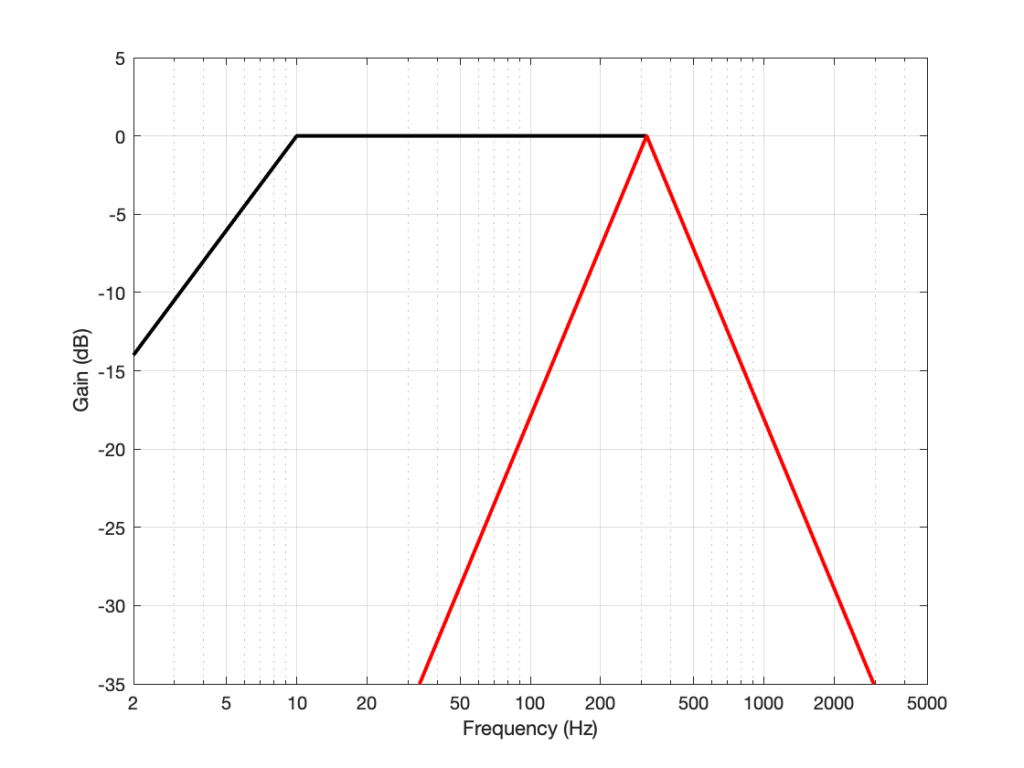The magnitude responses for the “Unweighted” (black) and “Weighted” filters for rumble measurements, specified in DIN 45 539

If the standard being used for the rumble measurement is the DIN 45 539 specification, then the resulting value is stated as the level difference between the measured filtered noise and a the standard output level, equivalent to the output when playing a 1 kHz tone with a lateral modulation velocity of 70.7 mm/sec. This detail is also worth noting, since it shows that the rumble value is a relative and not an absolute output level.

## Rotational speed

Every recording / playback system, whether for audio or for video signals, is based on the fundamental principle that the recording and the playback happen at the same rate. For example, a film that was recorded at 24 frames (or photos) per second (FPS) must also be played at 24 FPS to avoid objects and persons moving too slowly or too quickly. It’s also necessary that neither the recording nor the playback speed changes over time.

A phonographic LP is mastered with the intention that it will be played back at a rotational speed of 33 1/3 RPM (Revolutions Per Minute) or 45 RPM, depending on the disc. (These correspond to 1 revolution either every 1.8 seconds or every 1 1/3 seconds respectively.) We assume that the rotational speed of the lathe that was used to cut the master was both very accurate and very stable. Although it is the job of the turntable to duplicate this accuracy and stability as closely as possible, measurable errors occur for a number of reasons, both mechanical and electrical. When these errors are measured using especially-created audio signals like pure sine tones, the results are filtered and analyzed to give an impression of how audible they are when listening to music. However, a problem arises in that a simple specification (such as a single number for “Wow and Flutter”, for example) can only be correctly interpreted with the knowledge of how the value is produced.

### Accuracy

The first issue is the simple one of accuracy: is the turntable rotating the disc at the correct average speed? Most turntables have some kind of user control of this (both for the 33 and 45 RPM settings), since it will likely be necessary to adjust these occasionally over time, as the adjustment will drift with influences such as temperature and age.

### Stability

Like any audio system, regardless of whether it’s analogue or digital, the playback speed of the turntable will vary over time. As it increases and decreases, the pitch of the music at the output will increase and decrease proportionally. This is unavoidable. Therefore, there are two questions that result:

• How much does the speed change?
• What is the rate and pattern of the change?

In a turntable, the amount of the change in the rotational speed is directly proportional to the frequency shift in the audio output. Therefore for example, if the rotational speed decreases by 1% (for example, from 33 1/3 RPM to exactly 33 RPM), the audio output will drop in frequency by 1% (so a 440 Hz tone will be played as a 440 * 0.99 = 435.6 Hz tone). Whether this is audible is dependent on different factors including

• the rate of change to the new speed
(a 1% change 4 times a second is much easier to hear than a 1% change lasting 1 hour)
• the listener’s abilities
(for example, a person with “absolute pitch” may be able to recognise the change)
• the audio signal
(It is easier to detect a frequency shift of a single, long tone such as a note on a piano or pipe organ than it is of a short sound like a strike of claves or a sound with many enharmonic frequencies such as a snare drum.)

In an effort to simplify the specification of stability in analogue playback equipment such as turntables, four different classifications are used, each corresponding to different rates of change. These are drift, wow, flutter, and scrape, the two most popular of which are wow and flutter, and are typically grouped into one value to represent them.

### Drift

Frequency drift is the tendency of a playback device’s speed to change over time very slowly. Any variation that happens slower than once every 2 seconds (in other words, with a modulation frequency of less than 0.5 Hz) is considered to be drift. This is typically caused by changes such as temperature (as the playback device heats up) or variations in the power supply (due to changes in the mains supply, which can vary with changing loads throughout the day).

### Wow

Wow is a modulation in the speed ranging from once every 2 seconds to 6 times a second (0.5 Hz to 6 Hz). Note that, for a turntable, the rotational speed of the disc is within this range. (At 33 1/3 RPM: 1 revolution every 1.8 seconds is equal to approximately 0.556 Hz.)

### Flutter

Flutter describes a modulation in the speed ranging from 6 to 100 times a second (6 Hz to 100 Hz).

### Scrape

Scrape or scrape flutter describes changes in the speed that are higher than 100 Hz. This is typically only a problem with analogue tape decks (caused by the magnetic tape sticking and slipping on components in its path) and is not often used when classifying turntable performance.

### Measurement and Weighting

The easiest accurate method to measure the stability of the turntable’s speed within the range of Wow and Flutter is to follow one of the standard methods, of which there are many, but they are all similar. Examples of these standards are AES6-2008, CCIR 409-3, DIN 45507, and IEC-386. A special measurement disc containing a sine tone, usually with a frequency of 3150 Hz is played to a measurement device which then does a frequency analysis of the signal. In a perfect system, the result would be a 3150 Hz sine tone. In practice, however, the frequency of the tone varies over time, and it is this variation that is measured and analysed.

There is general agreement that we are particularly sensitive to a modulation in frequency of about 4 Hz (4 cycles per second) applied to many audio signals. As the modulation gets slower or faster, we are less sensitive to it, as was illustrated in the example above: (a 1% change 4 times a second is much easier to hear than a 1% change lasting 1 hour).

So, for example, if the analysis of the 3150 Hz tone shows that it varies by ±1% at a frequency of 4 Hz, then this will have a bigger impact on the result than if it varies by ±1% at a frequency of 0.1 Hz or 40 Hz. The amount of impact the measurement at any given modulation frequency has on the total result is shown as a “weighting curve” in the figure below.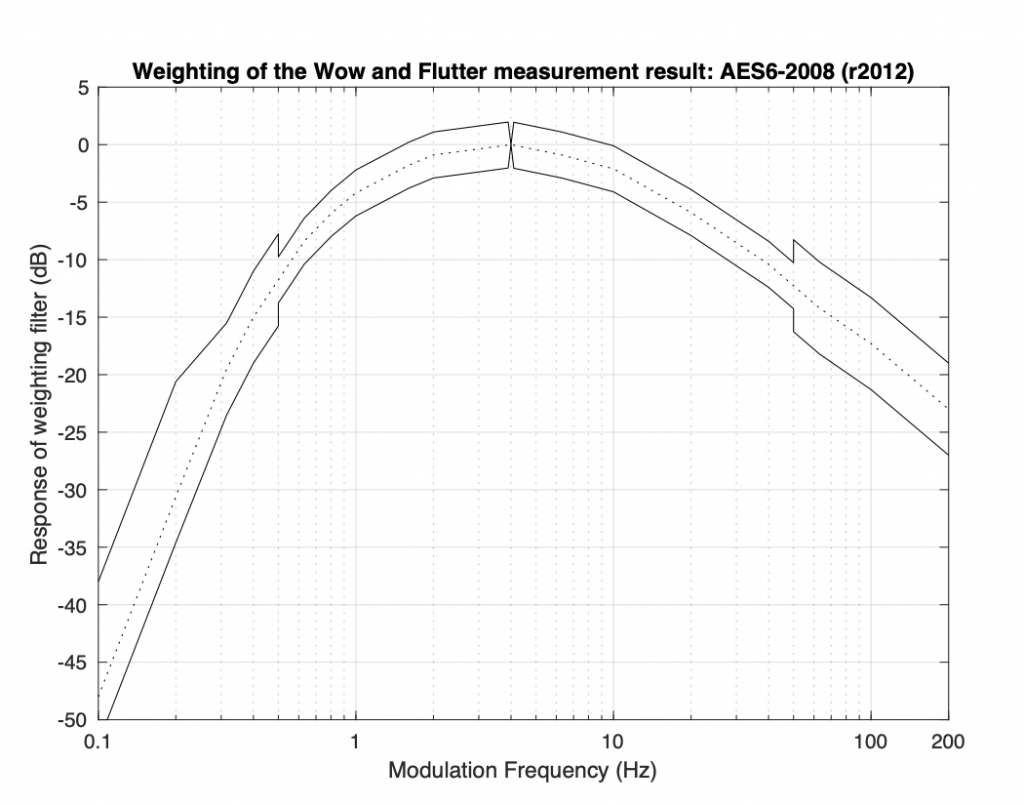Weighting applied to the Wow and Flutter measurement in most standard methods. See the text for an explanation.

As can be seen in this curve, a modulation at 4 Hz has a much bigger weight (or impact) on the final result than a modulation at 0.315 Hz or at 140 Hz, where a 20 dB attenuation is applied to their contribution to the total result. Since attenuating a value by 20 dB is the same as dividing it by 10; a ±1% modulation of the 3150Hz tone at 4 Hz will produce the same result as a ±10% modulation of the 3150 Hz tone at 140 Hz, for example.

This shows just one example of why comparing one Wow and Flutter measurement value should be interpreted very cautiously.

### Expressing the result

When looking at a Wow and Flutter specification, one will see something like <0.1%, <0.05% (DIN), or <0.1% (AES6). Like any audio specification, if the details of the measurement type are not included, then the value is useless. For example, “W&F: <0.1%” means nothing, since there is no way to know which method was used to arrive at this value.(Similarly, a specification like “Frequency Range: 20 Hz to 20 kHz” means nothing, since there is no information about the levels used to define the range.)

If the standard is included in the specification (DIN or AES6, for example), then it is still difficult to compare wow and flutter values. This is because, even when performing identical measurements and applying the same weighting curve shown in the figure above, there are different methods for arriving at the final value. The value that you see may be a peak value (the maximum deviation from the average speed), the peak-to-peak value (the difference between the minimum and the maximum speeds), the RMS (a version of the average deviation from the average speed), or something else.

The AES6-2008 standard, which is the currently accepted method of measuring and expressing the wow and flutter specification, uses a “2-sigma” method, which is a way of looking at the peak deviation to give a kind of “worst-case” scenario. In this method, the 3150 Hz tone is played from a disc and captured for as long a time as is possible or feasible. Firstly, the average value of the actual frequency of the output is found (in theory, it’s fixed at 3150 Hz, but this is never true). Next, the short-term variation of the actual frequency over time is compared to the average, and weighted using the filter shown above. The result shows the instantaneous frequency variations over the length of the captured signal, relative to the average frequency (however, the effect of very slow and very fast changes have been reduced by the filter). Finally, the standard deviation of the variation from the average is calculated, and multiplied by 2 (“2-Sigma”, or “two times the standard deviation”), resulting in the value that is shown as the specification. The reason two standard deviations is chosen is that (in the typical case where the deviation has a Gaussian distribution) the actual Wow & Flutter value should exceed this value no more than 5% of the time.

The reason this method is preferred today is that it uses a single number to express not only the wow and flutter, but the probability of the device reaching that value. For example, if a device is stated to have a “Wow and Flutter of <1% (AES6)”, then the actual deviation from the average speed will be less than 1% for 95% of the time you are listening to music. The principal reason this method was not used in the “old days” is that it requires statistical calculations applied to a signal that was captured from the output of the turntable, an option that was not available decades ago. The older DIN method that was used showed a long-term average level that was being measured in real-time using analogue equipment such as the device shown in below.

Unfortunately, however, it is still impossible to know whether a specification that reads “Wow and Flutter: 1% (AES6)” means 1% deviation with a modulation frequency of 4 Hz or 10% deviation with a modulation frequency of 140 Hz – or something else. It is also impossible to compare this value to a measurement done with one of the older standards such as the DIN method, for example.

# Turntables and Vinyl: Part 8

Back to Part 7

As was discussed in Part 3, when a record master is cut on a lathe, the cutter head follows a straight-line path as it moves from the outer rim to the inside of the disk. This means that it is always modulating in a direction that is perpendicular to the groove’s relative direction of travel, regardless of its distance from the centre.

A turntable should be designed to ensure that the stylus tracks the groove made by the cutter head in all aspects. This means that this perpendicular angle should be maintained across the entire surface of the disk. However, in the case of a tonearm that pivots, this is not possible, since the stylus follows a circular path, resulting in an angular tracking error.

The location of the pivot point, the tonearm’s shape, and the mounting of the cartridge can all contribute to reducing this error. Typically, tonearms are designed so that the cartridge is angled to not be in-line with the pivot point. This is done to ensure that there can be two locations on the record’s surface where the stylus is angled correctly relative to the groove.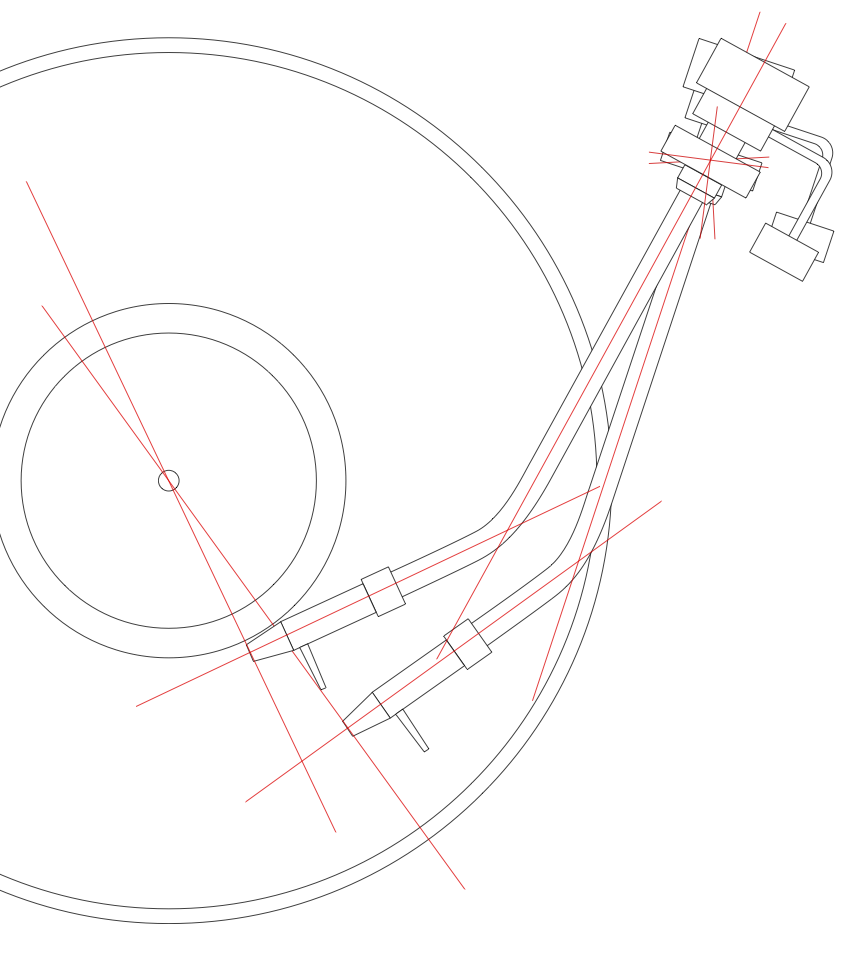A correctly-designed and aligned pivoting tonearm has a tracking error of 0º at only two locations on the disk.

However, the only real solution is to move the tonearm in a straight line across the disc, maintaining a position that is tangential to the groove, and therefore keeping the stylus located so that its movement is perpendicular to the groove’s relative direction of travel, just as it was with the cutter head on the lathe.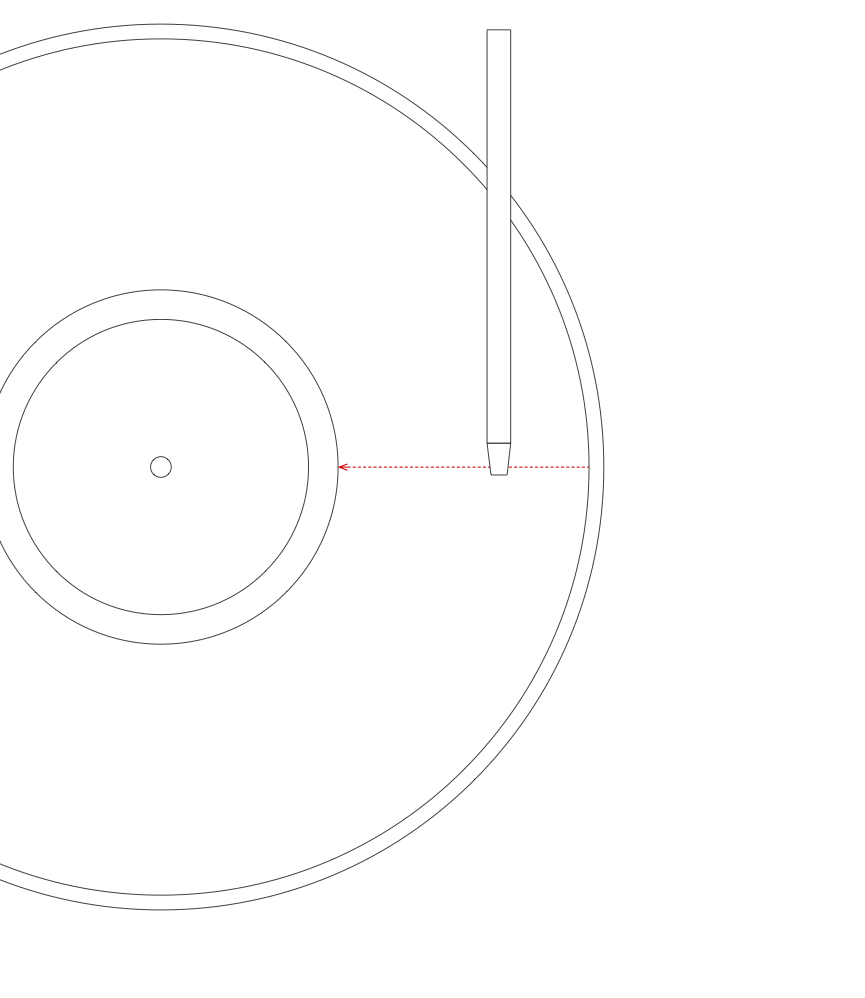A tonearm that travels sideways, maintaining an angle that is tangent to the groove at the stylus.

In a perfect system, the movement of the tonearm would be completely synchronous with the sideways “movement” of the groove underneath it, however, this is almost impossible to achieve. In the Beogram 4000c, a detection system is built into the tonearm that responds to the angular deviation from the resting position. The result is that the tonearm “wiggles” across the disk: the groove pulls the stylus towards the centre of the disk for a small distance before the detector reacts and moves the back of the tonearm to correct the angle.

Typically, the distance moved by the stylus before the detector engages the tracking motor is approximately 0.1 mm, which corresponds to a tracking error of approximately 0.044º.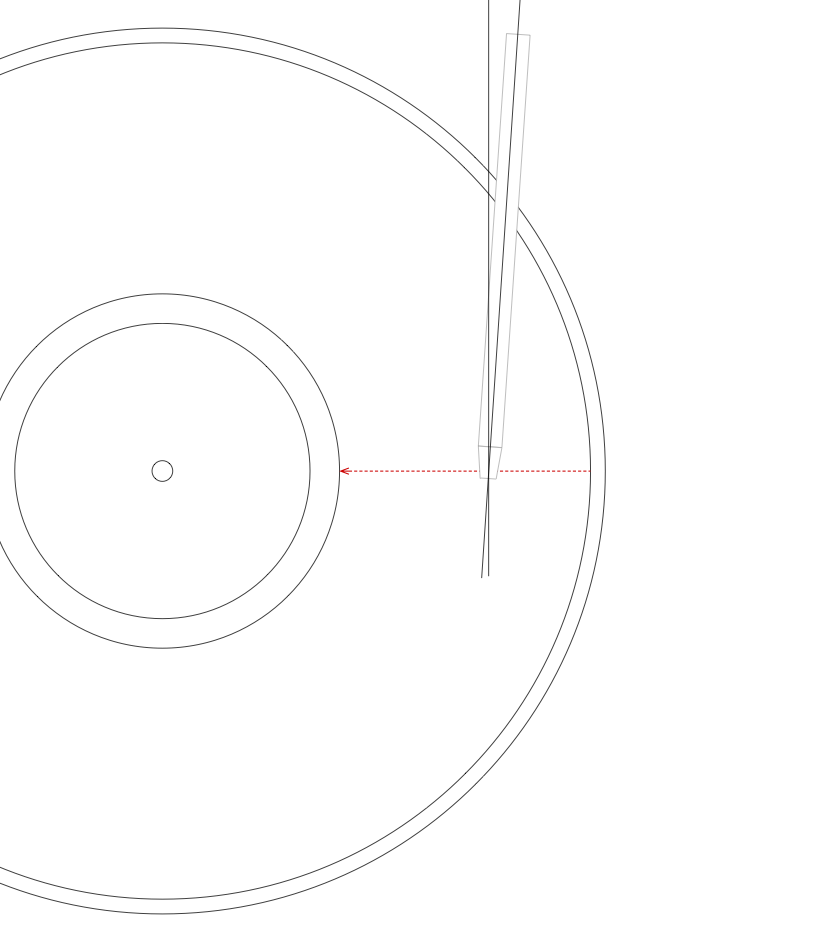An exaggerated representation of the maximum tracking error of the tonearm before the detector engages and corrects.

One of the primary artefacts caused by an angular tracking error is distortion of the audio signal: mainly second-order harmonic distortion of sinusoidal tones, and intermodulation distortion on more complex signals. (see “Have Tone Arm Designers Forgotten Their High-School Geometry?” in The Audio Critic, 1:31, Jan./Feb., 1977.) It can be intuitively understood that the distortion is caused by the fact that the stylus is being moved at a different angle than that for which it was designed.

It is possible to calculate an approximate value for this distortion level using the following equation:$Hd \approx 100 * \frac{ \omega A \alpha }{\omega_r R }$

Where$Hd$ is the harmonic distortion in percent,$\omega$ is the angular frequency of the modulation caused by the audio signal (calculated using$\omega = 2 \pi F$),$A$ is the peak amplitude in mm,$\alpha$ is the tracking error in degrees,$\omega_r$ is the angular frequency of rotation (the speed of the record in radians per second. For example, at 33 1/3 RPM,$\omega_r = 2 \pi 0.556 rev/sec = 3.49$) and$R$ is the radius (the distance of the groove from the centre of the disk). (see “Tracking Angle in Phonograph Pickups” by B.B. Bauer, Electronics (March 1945))

This equation can be re-written, separating the audio signal from the tonearm behaviour, as shown below.$Hd \approx 100 * \frac{ \omega A }{\omega_r} * \frac{\alpha}{R}$

which shows that, for a given audio frequency and disk rotation speed, the audio signal distortion is proportional to the horizontal tracking error over the distance of the stylus to the centre of the disk. (This is the reason one philosophy in the alignment of a pivoting tonearm is to ensure that the tracking error is reduced when approaching the centre of the disk, since the smaller the radius, the greater the distortion.)

It may be confusing as to why the position of the groove on the disk (the radius) has an influence on this value. The reason is that the distortion is dependent on the wavelength of the signal encoded in the groove. The longer the wavelength, the lower the distortion. As was shown in Figure 1 in Part 6 of this series, the wavelength of a constant frequency is longer on the outer groove of the disk than on the inner groove.

Using the Beogram 4000c as an example at its worst-case tracking error of 0.044º: if we have a 1 kHz sine wave with a modulation velocity of 34.1 mm/sec on a 33 1/3 RPM LP on the inner-most groove then the resulting 2nd-harmonic distortion will be 0.7% or about -43 dB relative to the signal. At the outer-most groove (assuming all other variables remain constant), the value will be roughly half of that, at 0.3% or -50 dB.